# Chapter 6 The Relational Algebra and Relational Calculus

• Slides: 93
Download presentationChapter 6 The Relational Algebra and Relational Calculus Copyright © 2011 Pearson Education, Inc. Publishing as Pearson Addison-Wesley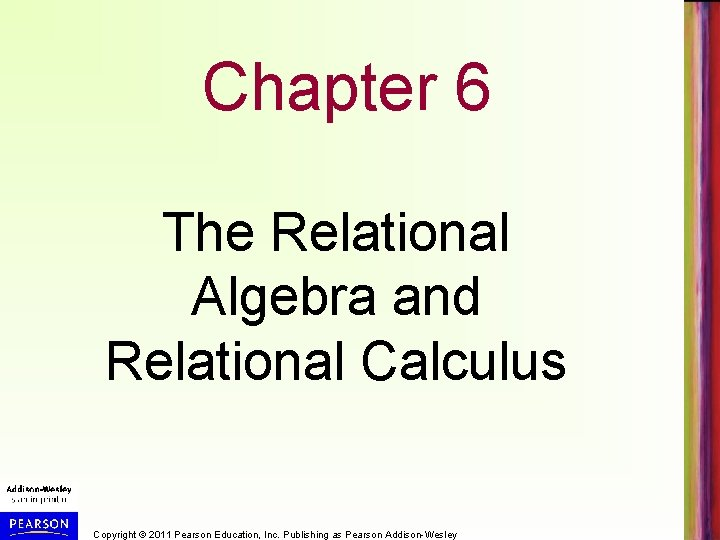Chapter 6 The Relational Algebra and Relational Calculus Copyright © 2011 Pearson Education, Inc. Publishing as Pearson Addison-WesleyChapter Outline • Relational Algebra – – – Unary Relational Operations Relational Algebra Operations From Set Theory Binary Relational Operations Additional Relational Operations Examples of Queries in Relational Algebra Relating SQL and Relational Algebra • Relational Calculus – Tuple Relational Calculus – Relating Tuple Relational Calculus to SQL – Domain Relational Calculus Copyright © 2011 Pearson Education, Inc. Publishing as Pearson Addison-WesleyRelational Algebra Overview • Relational algebra is the basic set of operations for theoretical relational model presented in Chapter 3 • SQL (chapters 4 and 5) is the standard language for practical relational databases • Relational algebra operations are used in DBMS query optimization – SQL is converted to relational operations for optimization and processing (see chapter 19) Copyright © 2011 Pearson Education, Inc. Publishing as Pearson Addison-Wesley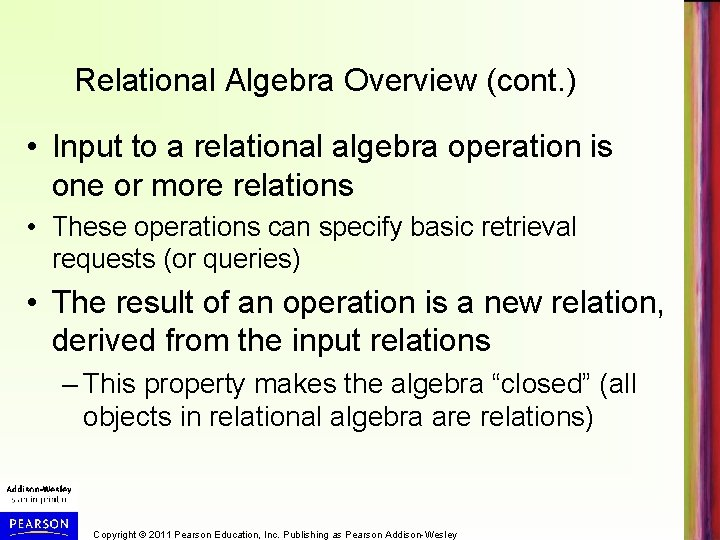Relational Algebra Overview (cont. ) • Input to a relational algebra operation is one or more relations • These operations can specify basic retrieval requests (or queries) • The result of an operation is a new relation, derived from the input relations – This property makes the algebra “closed” (all objects in relational algebra are relations) Copyright © 2011 Pearson Education, Inc. Publishing as Pearson Addison-WesleyRelational Algebra Overview (cont. ) • A query will typically require multiple operations – Result of one operation can be further manipulated using additional operations of the same algebra • A sequence of relational algebra operations forms a relational algebra expression – Final result is a relation that represents the result of a database query (or retrieval request) Copyright © 2011 Pearson Education, Inc. Publishing as Pearson Addison-WesleyRelational Algebra Overview (cont. ) • Relational Algebra consists of several groups of operations – Unary Relational Operations – one input table • SELECT (symbol: (sigma)) • PROJECT (symbol: (pi)) • RENAME (symbol: (rho)) – Relational Algebra Operations From Set Theory • UNION ( ), INTERSECTION ( ), DIFFERENCE (or MINUS, – ) • CARTESIAN PRODUCT ( x ) – Binary Relational Operations – two input tables • JOIN (several variations of JOIN exist) • DIVISION – Additional Relational Operations • OUTER JOINS, OUTER UNION • AGGREGATE FUNCTIONS (These compute summary of information: for example, SUM, COUNT, AVG, MIN, MAX) • Other operations Copyright © 2011 Pearson Education, Inc. Publishing as Pearson Addison-WesleyDatabase State for COMPANY • Examples in slides refer to the COMPANY database schema (Figure 3. 7 (shown on next slide)) • Examples of results of relational operations refer to the database state in Figure 3. 6 – shown after schema Copyright © 2011 Pearson Education, Inc. Publishing as Pearson Addison-Wesley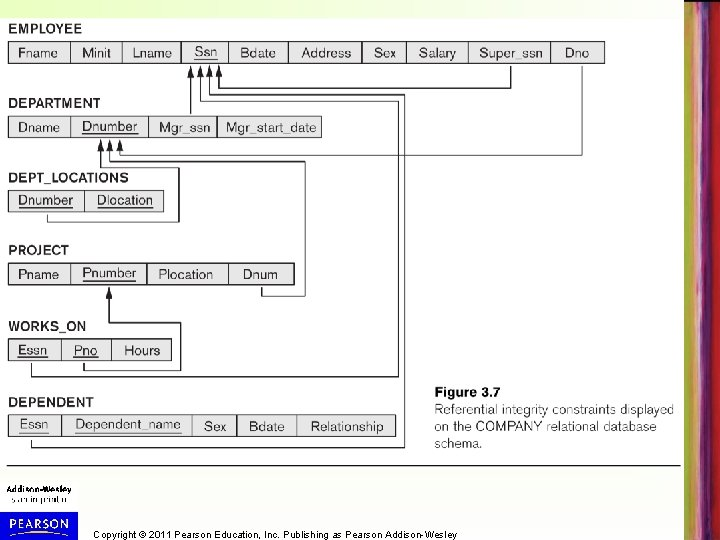Copyright © 2011 Pearson Education, Inc. Publishing as Pearson Addison-Wesley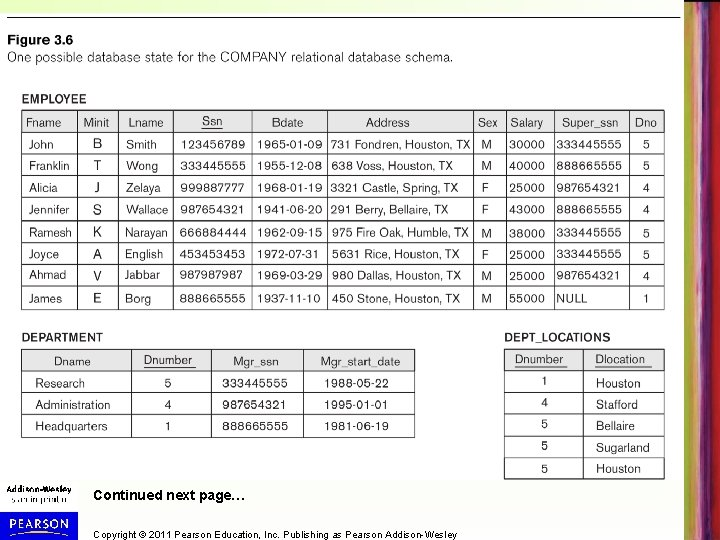Continued next page… Copyright © 2011 Pearson Education, Inc. Publishing as Pearson Addison-Wesley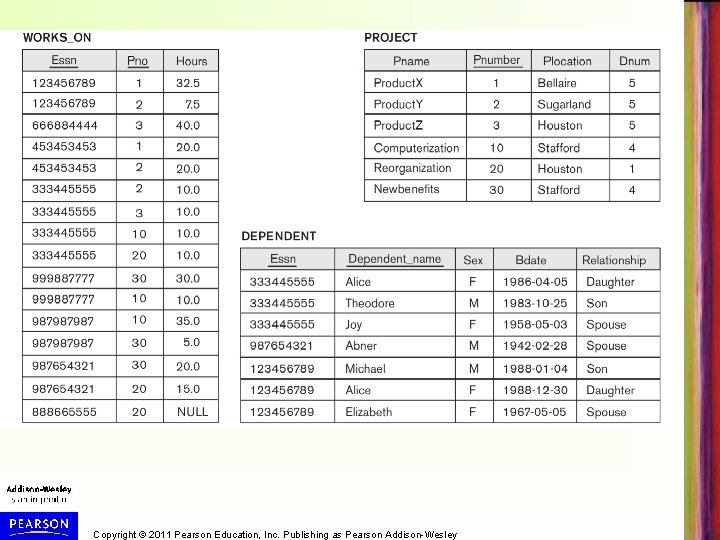Copyright © 2011 Pearson Education, Inc. Publishing as Pearson Addison-Wesley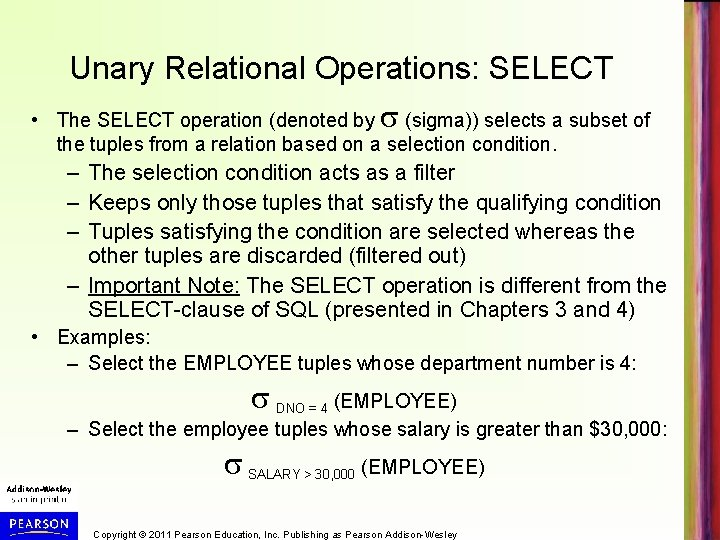Unary Relational Operations: SELECT • The SELECT operation (denoted by (sigma)) selects a subset of the tuples from a relation based on a selection condition. – The selection condition acts as a filter – Keeps only those tuples that satisfy the qualifying condition – Tuples satisfying the condition are selected whereas the other tuples are discarded (filtered out) – Important Note: The SELECT operation is different from the SELECT-clause of SQL (presented in Chapters 3 and 4) • Examples: – Select the EMPLOYEE tuples whose department number is 4: DNO = 4 (EMPLOYEE) – Select the employee tuples whose salary is greater than \$30, 000: SALARY > 30, 000 (EMPLOYEE) Copyright © 2011 Pearson Education, Inc. Publishing as Pearson Addison-Wesley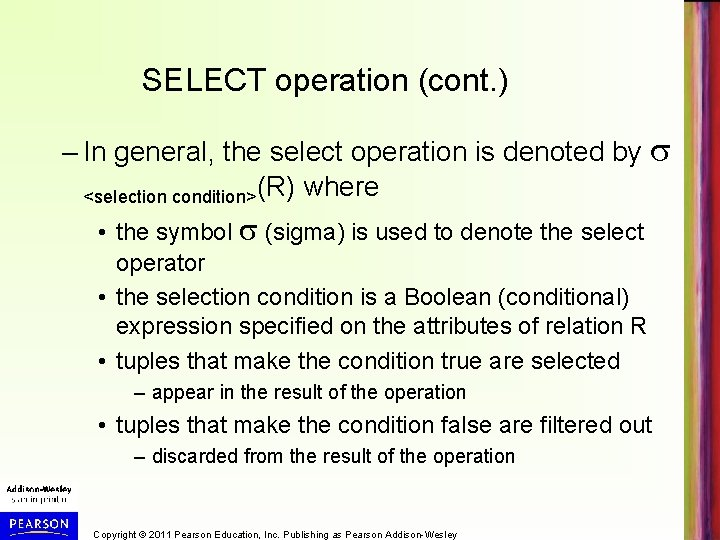SELECT operation (cont. ) – In general, the select operation is denoted by <selection condition>(R) where • the symbol (sigma) is used to denote the select operator • the selection condition is a Boolean (conditional) expression specified on the attributes of relation R • tuples that make the condition true are selected – appear in the result of the operation • tuples that make the condition false are filtered out – discarded from the result of the operation Copyright © 2011 Pearson Education, Inc. Publishing as Pearson Addison-WesleySELECT operation (cont. ) • SELECT Operation Properties – SELECT operation <selection condition>(R) produces a relation S – – that has the same schema (same attributes) as R SELECT is commutative: • <condition 1>( < condition 2> (R)) = <condition 2> ( < condition 1> (R)) Because of commutativity property, a cascade (sequence) of SELECT operations may be applied in any order: • <cond 1>( <cond 2> ( <cond 3> (R)) = <cond 2> ( <cond 3> <cond 1> ( R))) A cascade of SELECT operations may be replaced by a single selection with a conjunction of all the conditions: • <cond 1>( < cond 2> ( <cond 3>(R)) = <cond 1> AND < cond 2> AND < cond 3>(R))) The number of tuples in the result of a SELECT is less than (or equal to) the number of tuples in the input relation R Copyright © 2011 Pearson Education, Inc. Publishing as Pearson Addison-WesleyUnary Relational Operations: PROJECT • PROJECT Operation is denoted by (pi symbol) • Keeps certain columns (attributes) from a relation and discards the other columns. – PROJECT creates a vertical partitioning • The list of specified columns (attributes) is kept in each tuple • The other attributes in each tuple are discarded • Example: List each employee’s first and last name and salary: LNAME, FNAME, SALARY(EMPLOYEE) Copyright © 2011 Pearson Education, Inc. Publishing as Pearson Addison-Wesley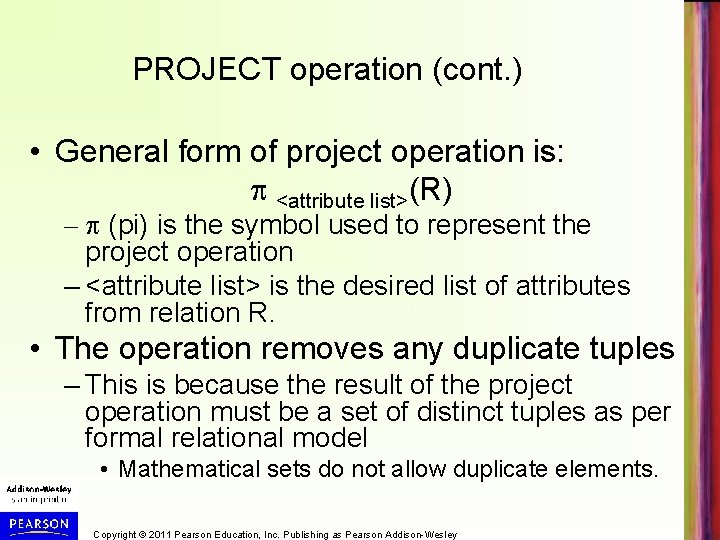PROJECT operation (cont. ) • General form of project operation is: <attribute list>(R) – (pi) is the symbol used to represent the project operation – <attribute list> is the desired list of attributes from relation R. • The operation removes any duplicate tuples – This is because the result of the project operation must be a set of distinct tuples as per formal relational model • Mathematical sets do not allow duplicate elements. Copyright © 2011 Pearson Education, Inc. Publishing as Pearson Addison-WesleyPROJECT operation (cont. ) • PROJECT Operation Properties – Number of tuples in result of projection <list>(R) less or equal to the number of tuples in R • If the list of projected attributes includes a key of R, then number of tuples in result of PROJECT is equal to the number of tuples in R – PROJECT is not commutative • <list 1> ( <list 2> (R) ) = <list 1> (R) as long as <list 2> contains the attributes in <list 1> Copyright © 2011 Pearson Education, Inc. Publishing as Pearson Addison-Wesley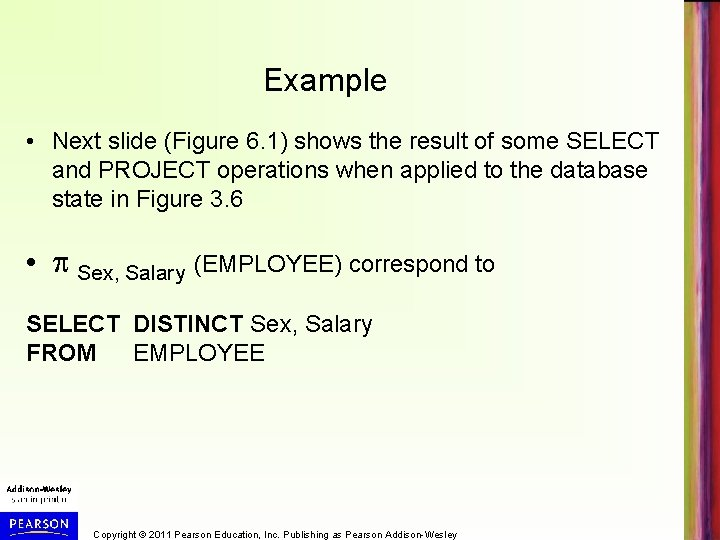Example • Next slide (Figure 6. 1) shows the result of some SELECT and PROJECT operations when applied to the database state in Figure 3. 6 • Sex, Salary (EMPLOYEE) correspond to SELECT DISTINCT Sex, Salary FROM EMPLOYEE Copyright © 2011 Pearson Education, Inc. Publishing as Pearson Addison-Wesley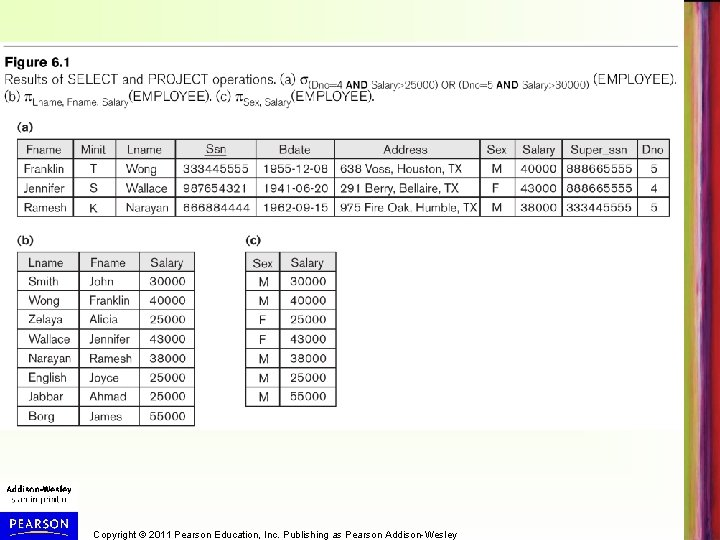Copyright © 2011 Pearson Education, Inc. Publishing as Pearson Addison-WesleyRelational Algebra Expressions • To apply several relational algebra operations – Either we write the operations as a single relational algebra expression by nesting the operations in parentheses, or – We apply one operation at a time and create intermediate result relations. • In the latter case, we must give names to the relations that hold the intermediate results. Copyright © 2011 Pearson Education, Inc. Publishing as Pearson Addison-WesleySingle expression versus sequence of relational operations (Example) • To retrieve the first name, last name, and salary of all employees who work in department number 5, we must apply a select and a project operation • Single relational algebra expression: – FNAME, LNAME, SALARY( DNO=5(EMPLOYEE)) • OR sequence of operations, giving a name to each intermediate relation: – DEP 5_EMPS <- DNO=5(EMPLOYEE) – RESULT <- FNAME, LNAME, SALARY (DEP 5_EMPS) Copyright © 2011 Pearson Education, Inc. Publishing as Pearson Addison-WesleyRelational SELECT and PROJECT Operations in SQL • The SELECT - clause of SQL lists the projection attributes, and the WHERE - clause includes the selection conditions • Example: The relational algebra expression: – FNAME, LNAME, SALARY( DNO=5(EMPLOYEE)) • Corresponds to the SQL query: SELECT FNAME, LNAME, SALARY FROM WHERE EMPLOYEE DNO=5 ; Copyright © 2011 Pearson Education, Inc. Publishing as Pearson Addison-Wesley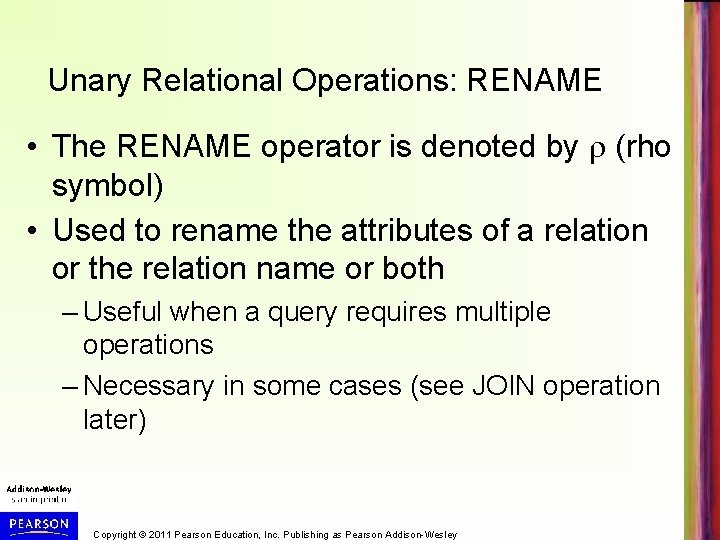Unary Relational Operations: RENAME • The RENAME operator is denoted by (rho symbol) • Used to rename the attributes of a relation or the relation name or both – Useful when a query requires multiple operations – Necessary in some cases (see JOIN operation later) Copyright © 2011 Pearson Education, Inc. Publishing as Pearson Addison-WesleyRENAME operation (cont. ) • The general RENAME operation can be expressed by any of the following forms: • S (B 1, B 2, …, Bn )(R) changes both: • the relation name to S, and • the column (attribute) names to B 1, …, Bn • S(R) changes: • the relation name only to S – (B 1, B 2, …, Bn )(R) changes: • the column (attribute) names only to B 1, …. . Bn Copyright © 2011 Pearson Education, Inc. Publishing as Pearson Addison-WesleyRENAME operation (cont. ) • For convenience, we also use shorthand for renaming an intermediate relation: – If we write: • R(FN, LN, SAL) FNAME, LNAME, SALARY (DEP 5_EMPS) • R will rename FNAME to FN, LNAME to LN, and SALARY to SAL • If we write: • R(F, M, L, S, B, A, SX, SAL, SU, D) EMPLOYEE • Then EMPLOYEE is renamed to R and the 10 attributes of EMPLOYEE are renamed to F, M, L, S, B, A, SX, SAL, SU, D, respectively Copyright © 2011 Pearson Education, Inc. Publishing as Pearson Addison-Wesley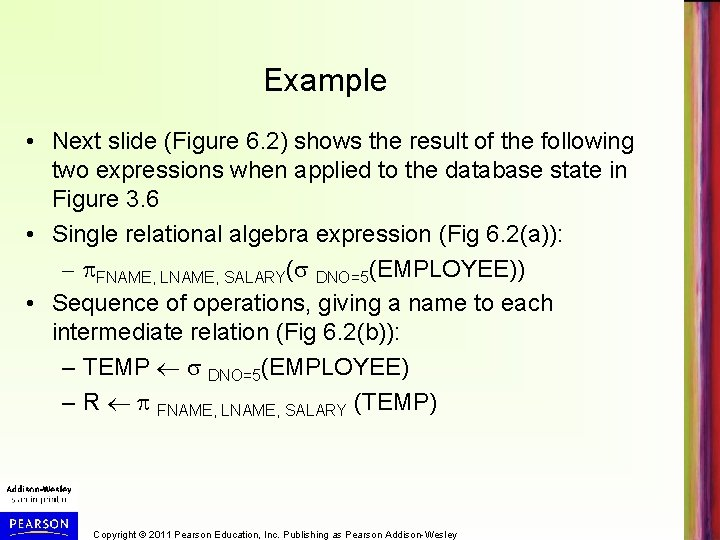Example • Next slide (Figure 6. 2) shows the result of the following two expressions when applied to the database state in Figure 3. 6 • Single relational algebra expression (Fig 6. 2(a)): – FNAME, LNAME, SALARY( DNO=5(EMPLOYEE)) • Sequence of operations, giving a name to each intermediate relation (Fig 6. 2(b)): – TEMP DNO=5(EMPLOYEE) – R FNAME, LNAME, SALARY (TEMP) Copyright © 2011 Pearson Education, Inc. Publishing as Pearson Addison-Wesley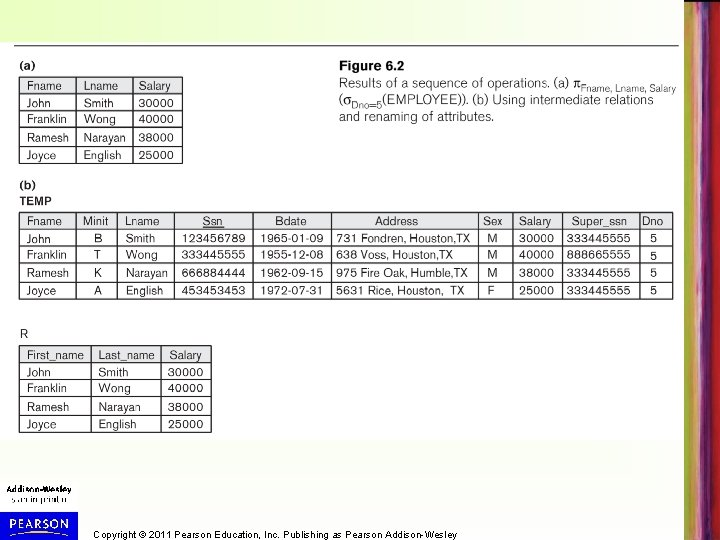Copyright © 2011 Pearson Education, Inc. Publishing as Pearson Addison-Wesley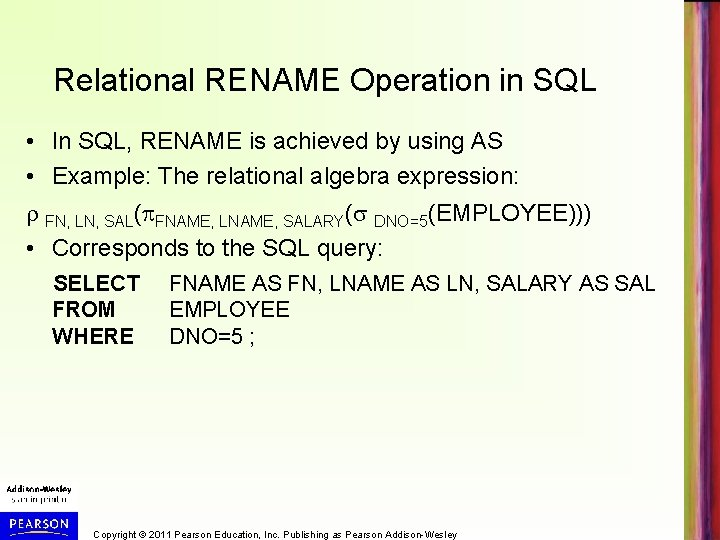Relational RENAME Operation in SQL • In SQL, RENAME is achieved by using AS • Example: The relational algebra expression: FN, LN, SAL( FNAME, LNAME, SALARY( DNO=5(EMPLOYEE))) • Corresponds to the SQL query: SELECT FNAME AS FN, LNAME AS LN, SALARY AS SAL FROM WHERE EMPLOYEE DNO=5 ; Copyright © 2011 Pearson Education, Inc. Publishing as Pearson Addison-WesleyRelational Algebra Operations from Set Theory: UNION • UNION Operation – Binary operation, denoted by – The result of R S, is a relation that includes all tuples that are either in R or in S or in both R and S – Duplicate tuples are eliminated – The two operand relations R and S must be “type compatible” (or “UNION compatible”) • R and S must have same number of attributes • Each pair of corresponding attributes must be type compatible (have same or compatible domains) Copyright © 2011 Pearson Education, Inc. Publishing as Pearson Addison-WesleyUNION operation (cont. ) • Example: – To retrieve the social security numbers of all employees who either work in department 5 (RESULT 1 below) or directly supervise an employee who works in department 5 (RESULT 2 below) – We can use the UNION operation as follows: DEP 5_EMPS DNO=5 (EMPLOYEE) RESULT 1 SSN(DEP 5_EMPS) RESULT 2(SSN) SUPERSSN(DEP 5_EMPS) RESULT 1 RESULT 2 – The union operation produces the tuples that are in either RESULT 1 or RESULT 2 or both (see Fig 6. 3) Copyright © 2011 Pearson Education, Inc. Publishing as Pearson Addison-Wesley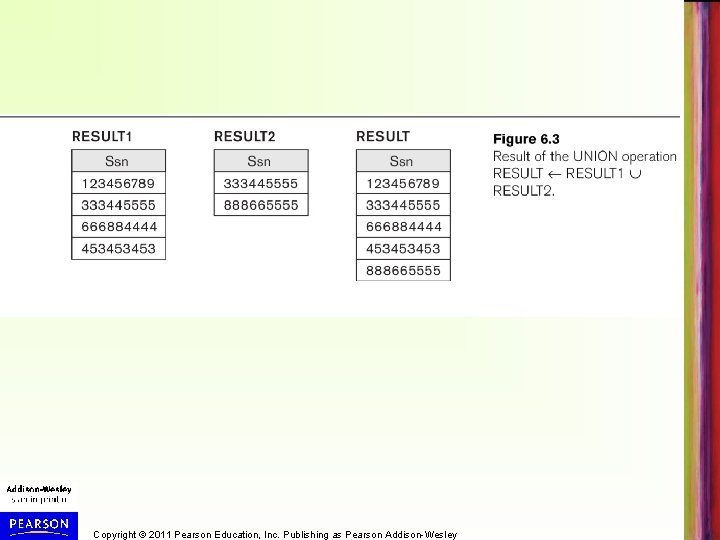Copyright © 2011 Pearson Education, Inc. Publishing as Pearson Addison-WesleyType Compatibility • Type Compatibility of operands is required for the binary set operation UNION , (also for INTERSECTION , and SET DIFFERENCE –, see next slides) • R 1(A 1, A 2, . . . , An) and R 2(B 1, B 2, . . . , Bn) are type compatible if: – they have the same number of attributes, and – the domains of corresponding attributes are type compatible (i. e. dom(Ai)=dom(Bi) for i=1, 2, . . . , n). • The resulting relation for R 1 R 2 (also for R 1 R 2, or R 1–R 2, see next slides) has the same attribute names as the first operand relation R 1 (by convention) Copyright © 2011 Pearson Education, Inc. Publishing as Pearson Addison-Wesley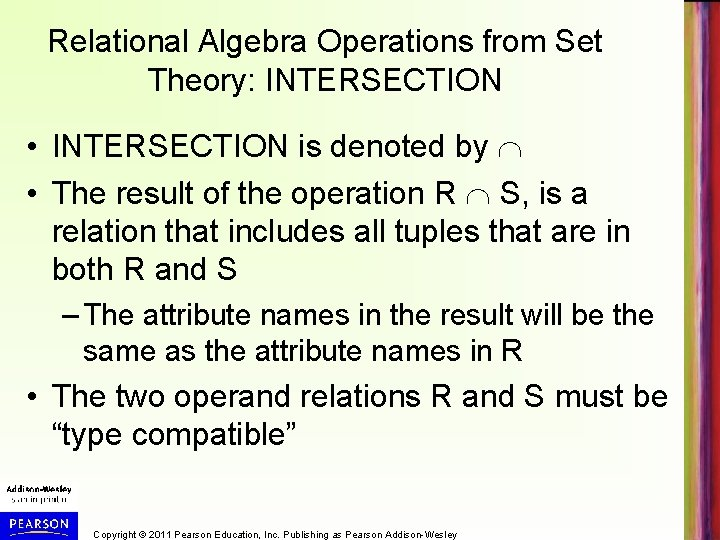Relational Algebra Operations from Set Theory: INTERSECTION • INTERSECTION is denoted by • The result of the operation R S, is a relation that includes all tuples that are in both R and S – The attribute names in the result will be the same as the attribute names in R • The two operand relations R and S must be “type compatible” Copyright © 2011 Pearson Education, Inc. Publishing as Pearson Addison-WesleyRelational Algebra Operations from Set Theory: SET DIFFERENCE • SET DIFFERENCE (also called MINUS or EXCEPT) is denoted by – • The result of R – S, is a relation that includes all tuples that are in R but not in S – The attribute names in the result will be the same as the attribute names in R • The two operand relations R and S must be “type compatible” Copyright © 2011 Pearson Education, Inc. Publishing as Pearson Addison-Wesley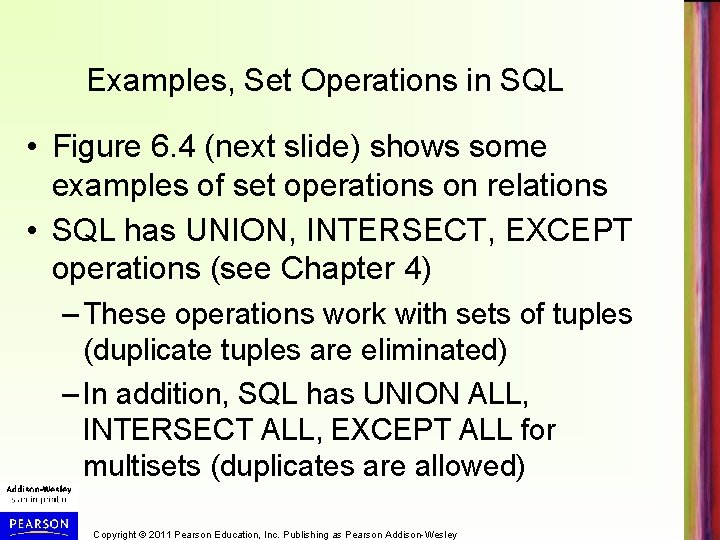Examples, Set Operations in SQL • Figure 6. 4 (next slide) shows some examples of set operations on relations • SQL has UNION, INTERSECT, EXCEPT operations (see Chapter 4) – These operations work with sets of tuples (duplicate tuples are eliminated) – In addition, SQL has UNION ALL, INTERSECT ALL, EXCEPT ALL for multisets (duplicates are allowed) Copyright © 2011 Pearson Education, Inc. Publishing as Pearson Addison-Wesley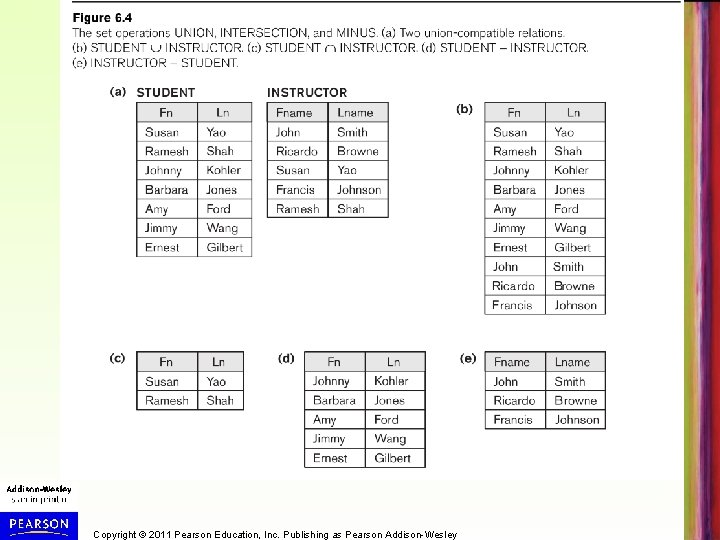Copyright © 2011 Pearson Education, Inc. Publishing as Pearson Addison-WesleySome properties of UNION, INTERSECT, and DIFFERENCE • Both union and intersection are commutative operations; that is – R S = S R, and R S = S R • Both union and intersection can be treated as n-ary operations applicable to any number of relations as both are associative operations; that is – R (S T) = (R S) T – (R S) T = R (S T) • The minus operation is not commutative; that is, in general – R – S ≠ S – R Copyright © 2011 Pearson Education, Inc. Publishing as Pearson Addison-WesleyRelational Algebra Operations from Set Theory: CARTESIAN PRODUCT • CARTESIAN (or CROSS) PRODUCT Operation – Different from the other three set operations – Used to combine tuples from two relations in a combinatorial fashion. – Denoted by R(A 1, A 2, . . . , An) x S(B 1, B 2, . . . , Bm) – Result is a relation Q with degree n + m attributes: • Q(A 1, A 2, . . . , An, B 1, B 2, . . . , Bm), in that order. – The resulting relation state has one tuple for each combination of tuples—one from R and one from S. – Hence, if R has n. R tuples (denoted as |R| = n. R ), and S has n. S tuples, then R x S will have n. R * n. S tuples. – R and S do NOT have to be "type compatible” Copyright © 2011 Pearson Education, Inc. Publishing as Pearson Addison-Wesley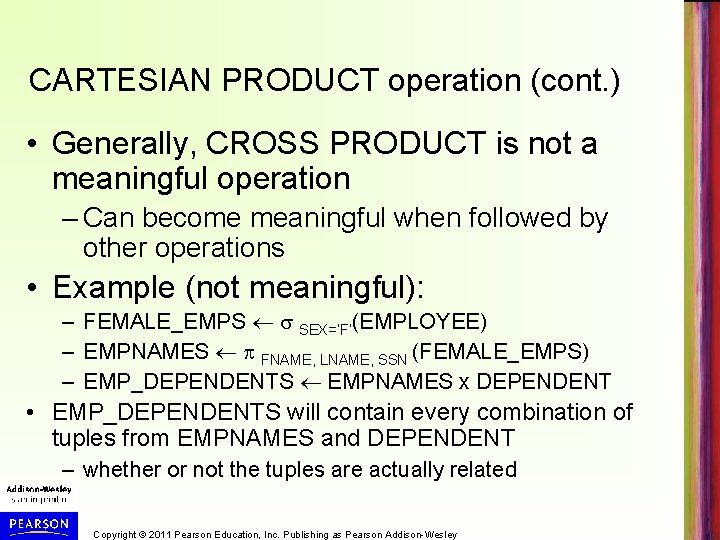CARTESIAN PRODUCT operation (cont. ) • Generally, CROSS PRODUCT is not a meaningful operation – Can become meaningful when followed by other operations • Example (not meaningful): – FEMALE_EMPS SEX=’F’(EMPLOYEE) – EMPNAMES FNAME, LNAME, SSN (FEMALE_EMPS) – EMP_DEPENDENTS EMPNAMES x DEPENDENT • EMP_DEPENDENTS will contain every combination of tuples from EMPNAMES and DEPENDENT – whether or not the tuples are actually related Copyright © 2011 Pearson Education, Inc. Publishing as Pearson Addison-Wesley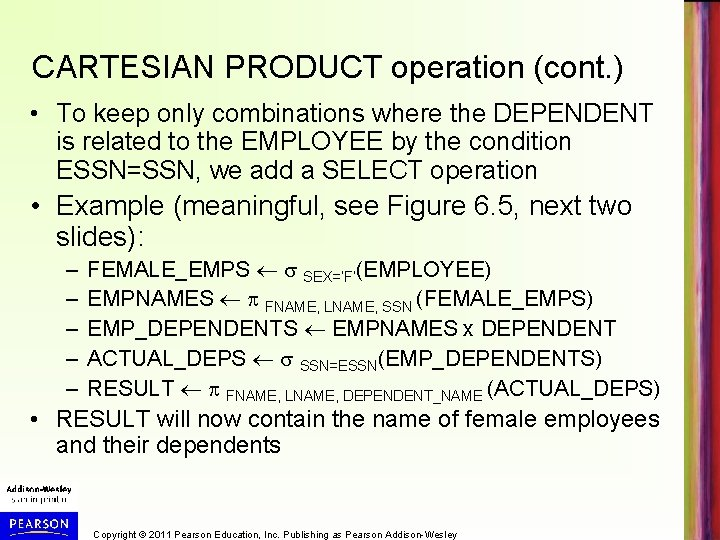CARTESIAN PRODUCT operation (cont. ) • To keep only combinations where the DEPENDENT is related to the EMPLOYEE by the condition ESSN=SSN, we add a SELECT operation • Example (meaningful, see Figure 6. 5, next two slides): – – – FEMALE_EMPS SEX=’F’(EMPLOYEE) EMPNAMES FNAME, LNAME, SSN (FEMALE_EMPS) EMP_DEPENDENTS EMPNAMES x DEPENDENT ACTUAL_DEPS SSN=ESSN(EMP_DEPENDENTS) RESULT FNAME, LNAME, DEPENDENT_NAME (ACTUAL_DEPS) • RESULT will now contain the name of female employees and their dependents Copyright © 2011 Pearson Education, Inc. Publishing as Pearson Addison-Wesley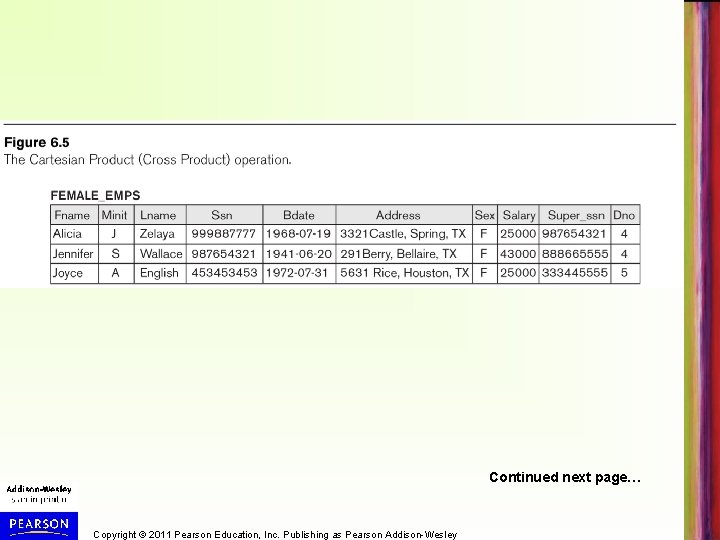Continued next page… Copyright © 2011 Pearson Education, Inc. Publishing as Pearson Addison-Wesley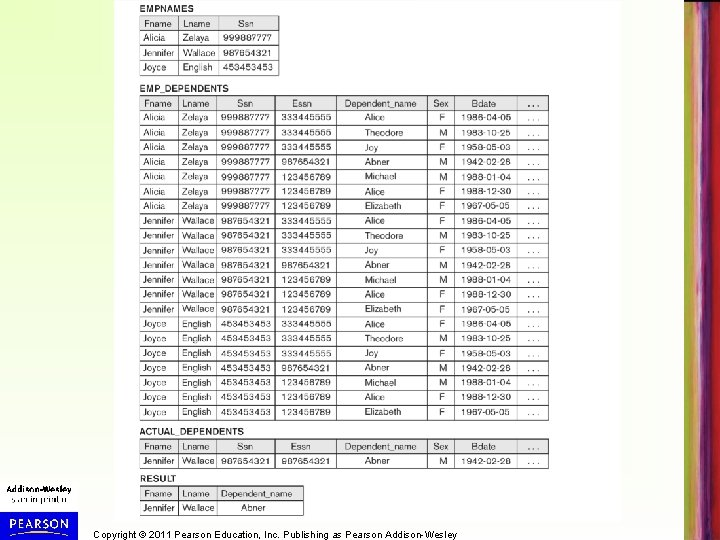Copyright © 2011 Pearson Education, Inc. Publishing as Pearson Addison-Wesley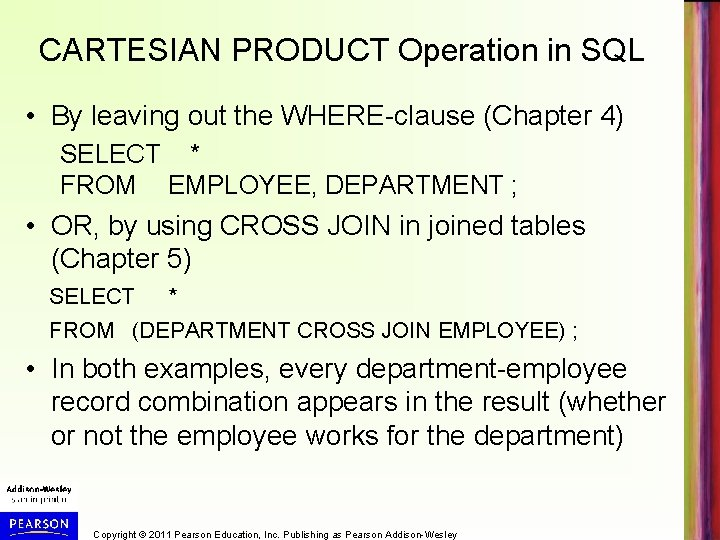CARTESIAN PRODUCT Operation in SQL • By leaving out the WHERE-clause (Chapter 4) SELECT * FROM EMPLOYEE, DEPARTMENT ; • OR, by using CROSS JOIN in joined tables (Chapter 5) SELECT * FROM (DEPARTMENT CROSS JOIN EMPLOYEE) ; • In both examples, every department-employee record combination appears in the result (whether or not the employee works for the department) Copyright © 2011 Pearson Education, Inc. Publishing as Pearson Addison-Wesley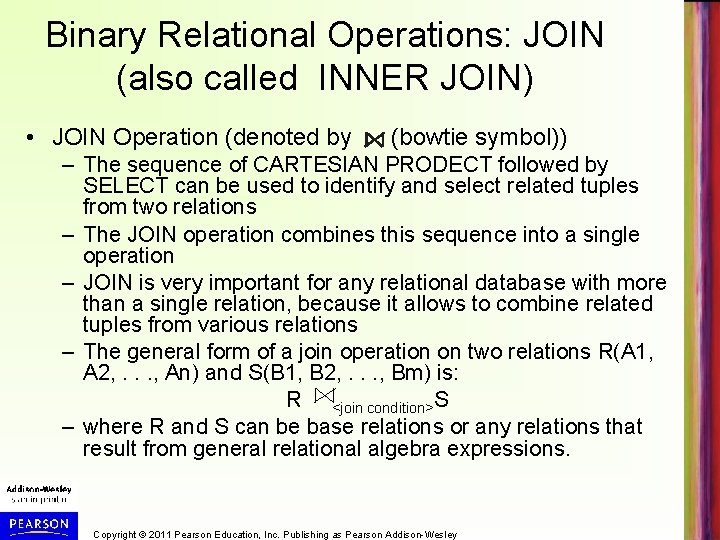Binary Relational Operations: JOIN (also called INNER JOIN) • JOIN Operation (denoted by (bowtie symbol)) – The sequence of CARTESIAN PRODECT followed by SELECT can be used to identify and select related tuples from two relations – The JOIN operation combines this sequence into a single operation – JOIN is very important for any relational database with more than a single relation, because it allows to combine related tuples from various relations – The general form of a join operation on two relations R(A 1, A 2, . . . , An) and S(B 1, B 2, . . . , Bm) is: R <join condition>S – where R and S can be base relations or any relations that result from general relational algebra expressions. Copyright © 2011 Pearson Education, Inc. Publishing as Pearson Addison-WesleyJOIN operation (cont. ) • Example: Suppose that we want to retrieve the name of the manager of each department. – To get the manager’s name, we need to combine each DEPARTMENT tuple with the EMPLOYEE tuple whose SSN value matches the MGRSSN value in the department tuple. – We do this by using the join operation – DEPT_MGR DEPARTMENT MGRSSN=SSN EMPLOYEE – RESULT Dname, Lname, Fname(DAPT_MGR) • MGRSSN=SSN is called the join condition – Combines each department record with the employee who manages the department – The join condition can also be specified as DEPARTMENT. MGRSSN= EMPLOYEE. SSN – Result in Figure 6. 6 (next slide) Copyright © 2011 Pearson Education, Inc. Publishing as Pearson Addison-Wesley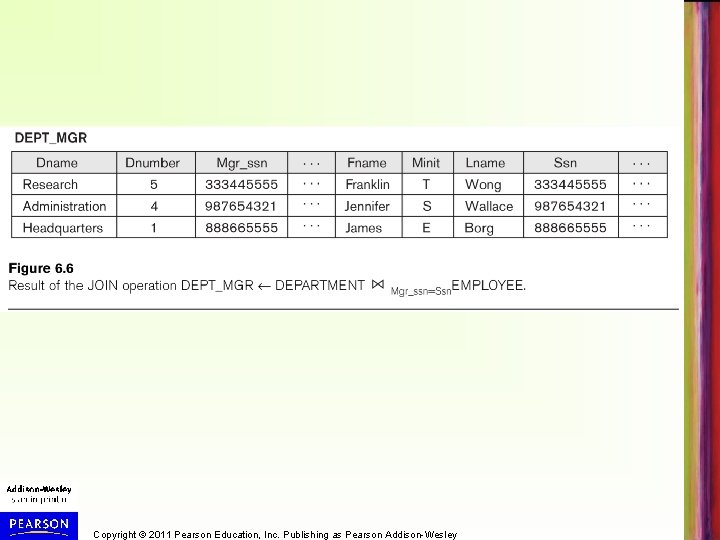Copyright © 2011 Pearson Education, Inc. Publishing as Pearson Addison-Wesley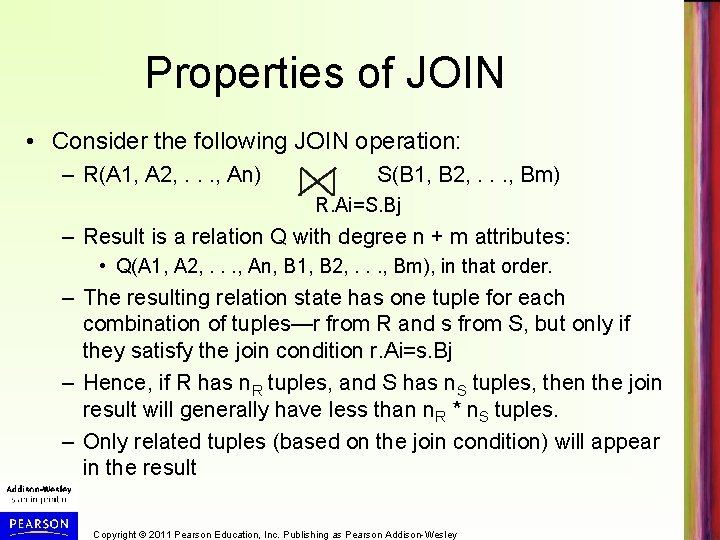Properties of JOIN • Consider the following JOIN operation: – R(A 1, A 2, . . . , An) S(B 1, B 2, . . . , Bm) R. Ai=S. Bj – Result is a relation Q with degree n + m attributes: • Q(A 1, A 2, . . . , An, B 1, B 2, . . . , Bm), in that order. – The resulting relation state has one tuple for each combination of tuples—r from R and s from S, but only if they satisfy the join condition r. Ai=s. Bj – Hence, if R has n. R tuples, and S has n. S tuples, then the join result will generally have less than n. R * n. S tuples. – Only related tuples (based on the join condition) will appear in the result Copyright © 2011 Pearson Education, Inc. Publishing as Pearson Addison-WesleyProperties of JOIN (cont. ) • General case of JOIN operation is called a Theta-join: R S theta • The join condition is called theta • Theta can be any general boolean expression on the attributes of R and S; for example: – R. Ai<S. Bj AND (R. Ak=S. Bl OR R. Ap<S. Bq) • Most join conditions involve one or more equality conditions “AND”ed together; for example: – R. Ai=S. Bj AND R. Ak=S. Bl AND R. Ap=S. Bq Copyright © 2011 Pearson Education, Inc. Publishing as Pearson Addison-Wesley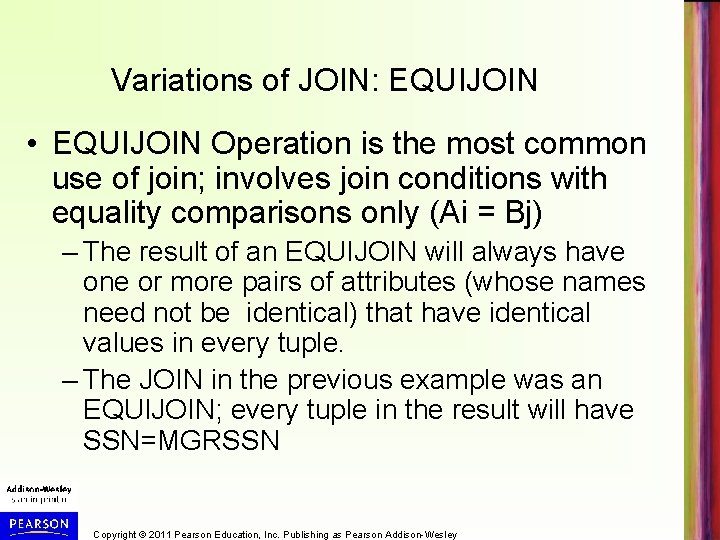Variations of JOIN: EQUIJOIN • EQUIJOIN Operation is the most common use of join; involves join conditions with equality comparisons only (Ai = Bj) – The result of an EQUIJOIN will always have one or more pairs of attributes (whose names need not be identical) that have identical values in every tuple. – The JOIN in the previous example was an EQUIJOIN; every tuple in the result will have SSN=MGRSSN Copyright © 2011 Pearson Education, Inc. Publishing as Pearson Addison-WesleyVariations of JOIN: NATURAL JOIN • NATURAL JOIN Operation – Another variation of JOIN called NATURAL JOIN — denoted by * — was created to get rid of the second (superfluous) attribute in an EQUIJOIN result. • because one of each pair of attributes with identical values is superfluous – The standard definition of natural join requires that the two join attributes, or each pair of corresponding join attributes, have the same name in both relations – If this is not the case, a renaming operation is applied first. Copyright © 2011 Pearson Education, Inc. Publishing as Pearson Addison-Wesley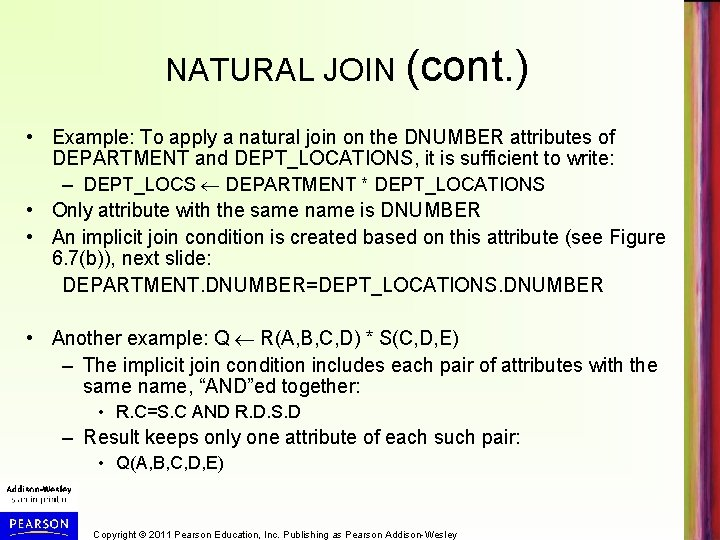NATURAL JOIN (cont. ) • Example: To apply a natural join on the DNUMBER attributes of DEPARTMENT and DEPT_LOCATIONS, it is sufficient to write: – DEPT_LOCS DEPARTMENT * DEPT_LOCATIONS • Only attribute with the same name is DNUMBER • An implicit join condition is created based on this attribute (see Figure 6. 7(b)), next slide: DEPARTMENT. DNUMBER=DEPT_LOCATIONS. DNUMBER • Another example: Q R(A, B, C, D) * S(C, D, E) – The implicit join condition includes each pair of attributes with the same name, “AND”ed together: • R. C=S. C AND R. D. S. D – Result keeps only one attribute of each such pair: • Q(A, B, C, D, E) Copyright © 2011 Pearson Education, Inc. Publishing as Pearson Addison-WesleyCopyright © 2011 Pearson Education, Inc. Publishing as Pearson Addison-Wesley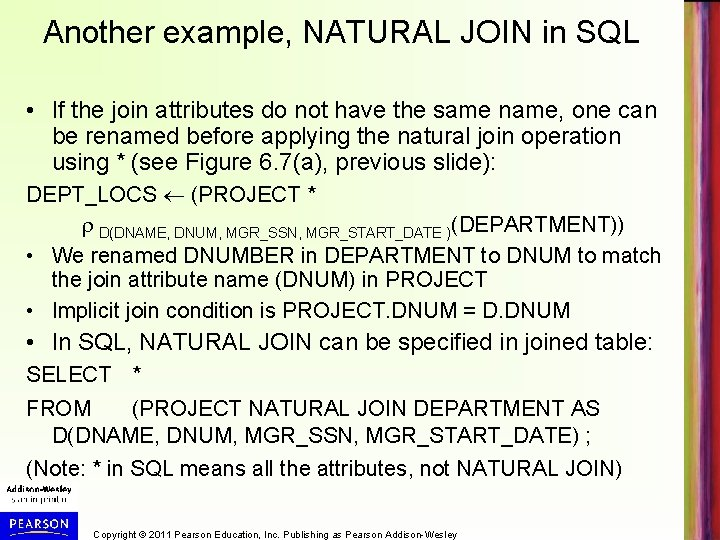Another example, NATURAL JOIN in SQL • If the join attributes do not have the same name, one can be renamed before applying the natural join operation using * (see Figure 6. 7(a), previous slide): DEPT_LOCS (PROJECT * D(DNAME, DNUM, MGR_SSN, MGR_START_DATE )(DEPARTMENT)) • We renamed DNUMBER in DEPARTMENT to DNUM to match the join attribute name (DNUM) in PROJECT • Implicit join condition is PROJECT. DNUM = D. DNUM • In SQL, NATURAL JOIN can be specified in joined table: SELECT * FROM (PROJECT NATURAL JOIN DEPARTMENT AS D(DNAME, DNUM, MGR_SSN, MGR_START_DATE) ; (Note: * in SQL means all the attributes, not NATURAL JOIN) Copyright © 2011 Pearson Education, Inc. Publishing as Pearson Addison-Wesley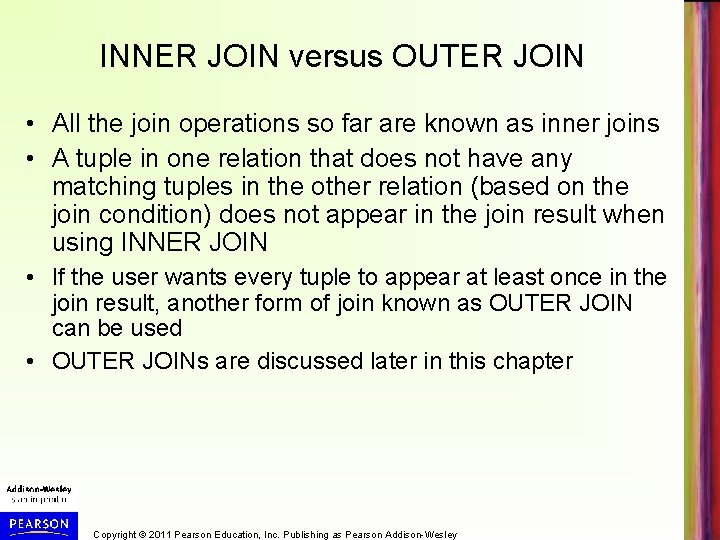INNER JOIN versus OUTER JOIN • All the join operations so far are known as inner joins • A tuple in one relation that does not have any matching tuples in the other relation (based on the join condition) does not appear in the join result when using INNER JOIN • If the user wants every tuple to appear at least once in the join result, another form of join known as OUTER JOIN can be used • OUTER JOINs are discussed later in this chapter Copyright © 2011 Pearson Education, Inc. Publishing as Pearson Addison-Wesley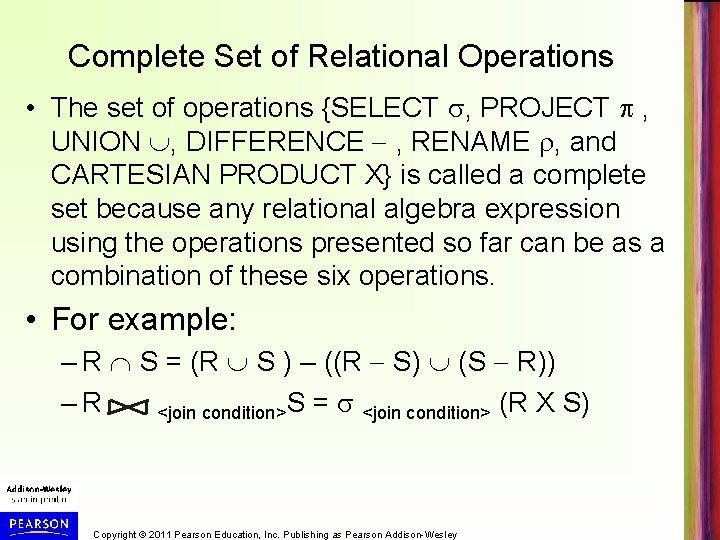Complete Set of Relational Operations • The set of operations {SELECT , PROJECT , UNION , DIFFERENCE - , RENAME , and CARTESIAN PRODUCT X} is called a complete set because any relational algebra expression using the operations presented so far can be as a combination of these six operations. • For example: – R S = (R S ) – ((R - S) (S - R)) – R <join condition>S = <join condition> (R X S) Copyright © 2011 Pearson Education, Inc. Publishing as Pearson Addison-Wesley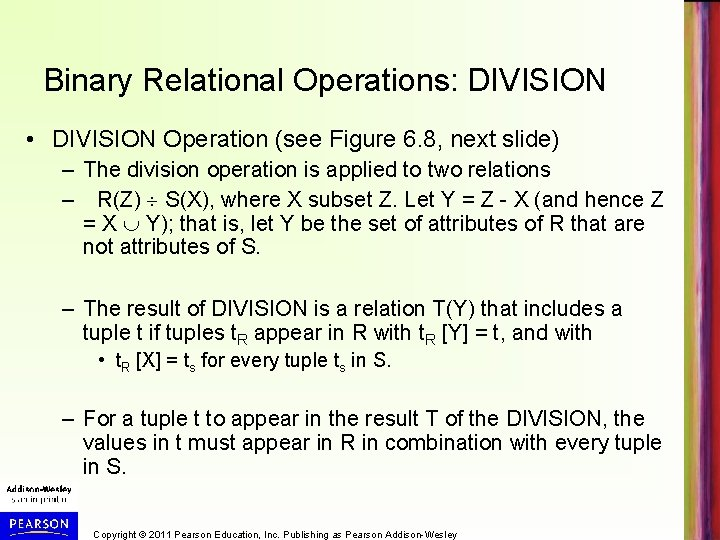Binary Relational Operations: DIVISION • DIVISION Operation (see Figure 6. 8, next slide) – The division operation is applied to two relations – R(Z) S(X), where X subset Z. Let Y = Z - X (and hence Z = X Y); that is, let Y be the set of attributes of R that are not attributes of S. – The result of DIVISION is a relation T(Y) that includes a tuple t if tuples t. R appear in R with t. R [Y] = t, and with • t. R [X] = ts for every tuple ts in S. – For a tuple t to appear in the result T of the DIVISION, the values in t must appear in R in combination with every tuple in S. Copyright © 2011 Pearson Education, Inc. Publishing as Pearson Addison-WesleyCopyright © 2011 Pearson Education, Inc. Publishing as Pearson Addison-Wesley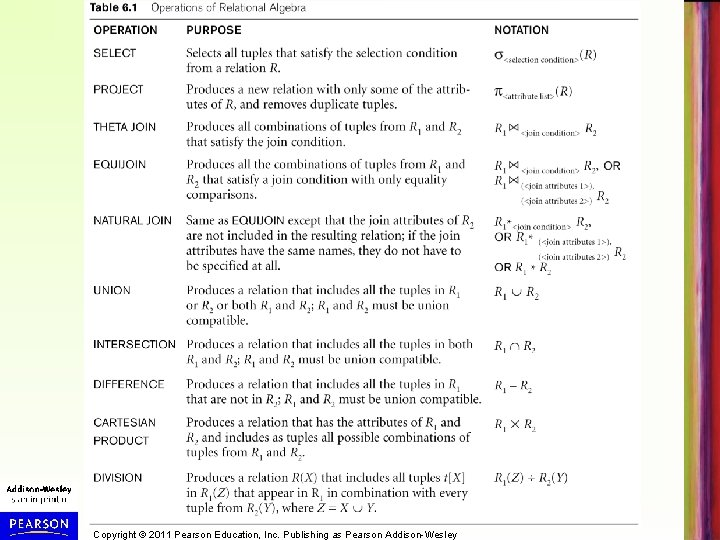Copyright © 2011 Pearson Education, Inc. Publishing as Pearson Addison-WesleyQuery Tree Notation • Query Tree (see Figure 6. 9, next slide) – An internal data structure to represent a query – Standard technique for estimating the work involved in executing the query, the generation of intermediate results, and the optimization of execution (see Chapter 19) – Nodes stand for operations like selection, projection, join, renaming, division, …. – Leaf nodes represent base relations – A tree gives a good visual feel of the complexity of the query and the operations involved – Algebraic Query Optimization consists of rewriting the query or modifying the query tree into an equivalent tree (see Chapter 19) Copyright © 2011 Pearson Education, Inc. Publishing as Pearson Addison-WesleyFor every project located in ‘Stafford’, list the project number, the controlling department number, and the department manager’s last name, address, and birth date. Pnumber, Dnum, Lname, Address, Bdate((( Plocation=‘Stafford’(PROJECT)) Dnum = Dnumber(DEPARTMENT)) Mgr_ssn=Ssn(EMPLOYEE)) Copyright © 2011 Pearson Education, Inc. Publishing as Pearson Addison-Wesley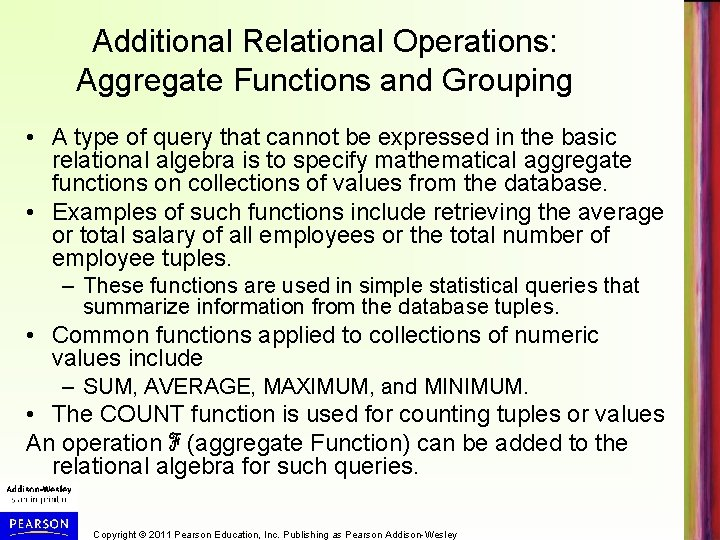Additional Relational Operations: Aggregate Functions and Grouping • A type of query that cannot be expressed in the basic relational algebra is to specify mathematical aggregate functions on collections of values from the database. • Examples of such functions include retrieving the average or total salary of all employees or the total number of employee tuples. – These functions are used in simple statistical queries that summarize information from the database tuples. • Common functions applied to collections of numeric values include – SUM, AVERAGE, MAXIMUM, and MINIMUM. • The COUNT function is used for counting tuples or values An operation ℱ (aggregate Function) can be added to the relational algebra for such queries. Copyright © 2011 Pearson Education, Inc. Publishing as Pearson Addison-WesleyAggregate Function Operation • Use of the Aggregate Function operation ℱ – ℱMAX Salary (EMPLOYEE) retrieves the maximum salary value from the EMPLOYEE relation – ℱMIN Salary (EMPLOYEE) retrieves the minimum Salary value from the EMPLOYEE relation – ℱSUM Salary (EMPLOYEE) retrieves the sum of all the Salary values from the EMPLOYEE relation – ℱCOUNT SSN, AVERAGE Salary (EMPLOYEE) computes the count (number) of employees and their average salary (Figure 6. 10(c)) • Note: COUNT(*) just counts the number of rows Copyright © 2011 Pearson Education, Inc. Publishing as Pearson Addison-WesleyUsing Grouping with Aggregation • The previous examples summarized one or more attributes for a set of tuples into a single tuple – Maximum Salary or Count (number of) Ssn • Grouping can be combined with Aggregate Functions • Example: For each department, retrieve the DNO, COUNT SSN, and AVERAGE SALARY • A variation of aggregate operation ℱ allows this: – Grouping attribute placed to left of symbol – Aggregate functions to right of symbol – DNO ℱCOUNT SSN, AVERAGE Salary (EMPLOYEE) • Above operation groups employees by DNO (department number) and computes the count of employees and average salary per department (see Figure 6. 10) Copyright © 2011 Pearson Education, Inc. Publishing as Pearson Addison-Wesley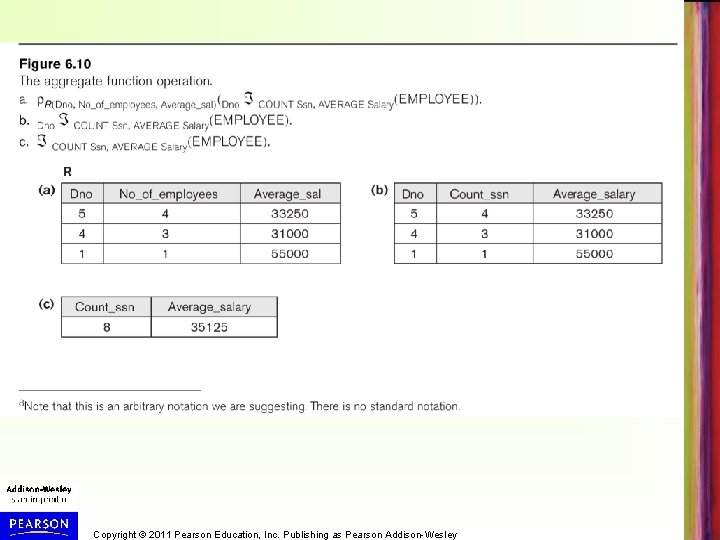Copyright © 2011 Pearson Education, Inc. Publishing as Pearson Addison-Wesley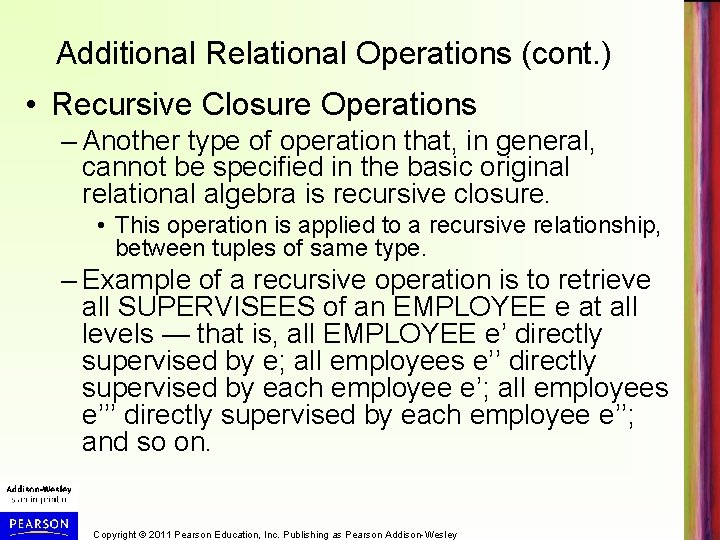Additional Relational Operations (cont. ) • Recursive Closure Operations – Another type of operation that, in general, cannot be specified in the basic original relational algebra is recursive closure. • This operation is applied to a recursive relationship, between tuples of same type. – Example of a recursive operation is to retrieve all SUPERVISEES of an EMPLOYEE e at all levels — that is, all EMPLOYEE e’ directly supervised by e; all employees e’’ directly supervised by each employee e’; all employees e’’’ directly supervised by each employee e’’; and so on. Copyright © 2011 Pearson Education, Inc. Publishing as Pearson Addison-Wesley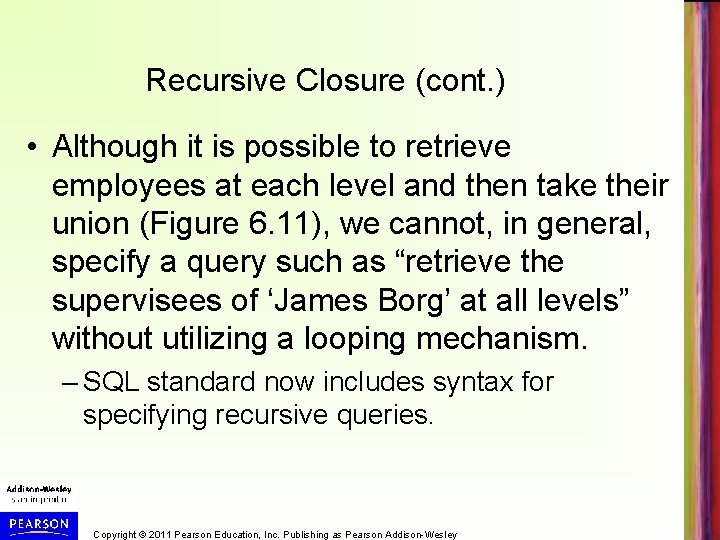Recursive Closure (cont. ) • Although it is possible to retrieve employees at each level and then take their union (Figure 6. 11), we cannot, in general, specify a query such as “retrieve the supervisees of ‘James Borg’ at all levels” without utilizing a looping mechanism. – SQL standard now includes syntax for specifying recursive queries. Copyright © 2011 Pearson Education, Inc. Publishing as Pearson Addison-Wesley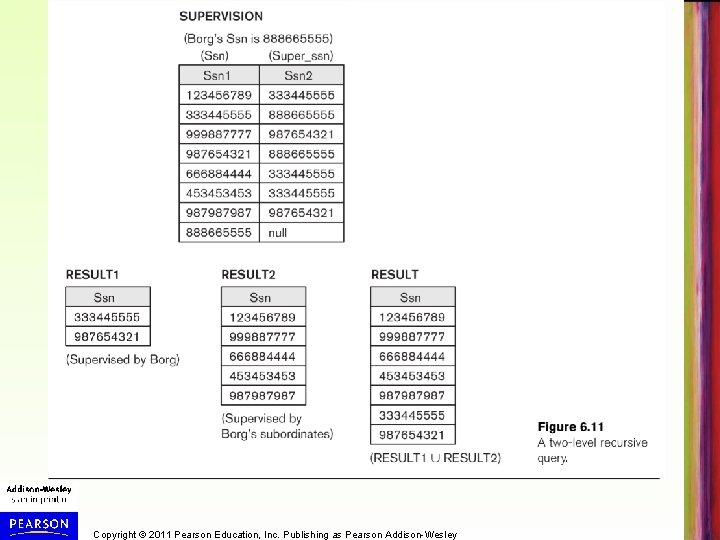Copyright © 2011 Pearson Education, Inc. Publishing as Pearson Addison-WesleyAdditional Relational Operations (cont. ) • The OUTER JOIN Operation – In NATURAL JOIN and EQUIJOIN, tuples without a matching (or related) tuple are eliminated from the join result • Tuples with null in the join attributes are also eliminated • Some user queries may want to see all tuples. – A set of operations, called OUTER joins, can be used when the user wants to keep all the tuples in R, or all those in S, or both in the result of the join (regardless of whether or not they have matching tuples in the other relation). Copyright © 2011 Pearson Education, Inc. Publishing as Pearson Addison-WesleyOUTER JOIN Operations (cont. ) • LEFT OUTER JOIN keeps every tuple in the first or left relation R in R S; if no matching tuple is found in S, then the attributes of S in the join result are filled or “padded” with null values. • RIGHT OUTER JOIN keeps every tuple in the second or right relation S in the result of R S. • FULL OUTER JOIN denoted by keeps all tuples in both the left and the right relations when no matching tuples are found, padding them with null values as needed. Copyright © 2011 Pearson Education, Inc. Publishing as Pearson Addison-WesleyLEFT OUTER JOIN Example • Retrieve the name of every employee, and if the employee manages a department, retrieve the name of that department. • Result is shown on next slide. DEPT_MGR EMPLOYEE SSN=MGR_SSN DEPARTMENT RESULT FNAME, MINIT, LNAME, DNAME(DEPT_MGR) Copyright © 2011 Pearson Education, Inc. Publishing as Pearson Addison-Wesley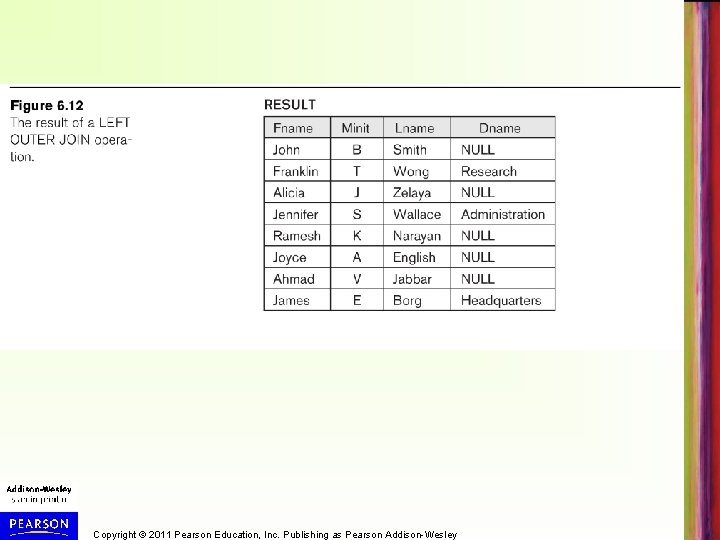Copyright © 2011 Pearson Education, Inc. Publishing as Pearson Addison-WesleyAdditional Relational Operations (cont. ) • OUTER UNION Operations – OUTER UNION takes the union of tuples from two relations that are not fully type compatible. – Union of tuples in two relations R(X, Y) and S(X, Z) that are partially compatible (only some of their attributes, say X, are type compatible). – The attributes X that are type compatible are represented only once in the result – Attributes that are not type compatible (Y and Z) are also kept in the result relation T(X, Y, Z). Copyright © 2011 Pearson Education, Inc. Publishing as Pearson Addison-Wesley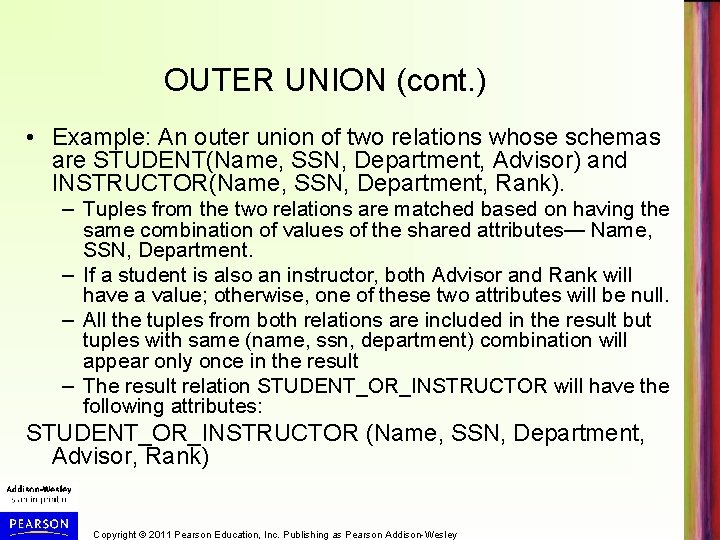OUTER UNION (cont. ) • Example: An outer union of two relations whose schemas are STUDENT(Name, SSN, Department, Advisor) and INSTRUCTOR(Name, SSN, Department, Rank). – Tuples from the two relations are matched based on having the same combination of values of the shared attributes— Name, SSN, Department. – If a student is also an instructor, both Advisor and Rank will have a value; otherwise, one of these two attributes will be null. – All the tuples from both relations are included in the result but tuples with same (name, ssn, department) combination will appear only once in the result – The result relation STUDENT_OR_INSTRUCTOR will have the following attributes: STUDENT_OR_INSTRUCTOR (Name, SSN, Department, Advisor, Rank) Copyright © 2011 Pearson Education, Inc. Publishing as Pearson Addison-Wesley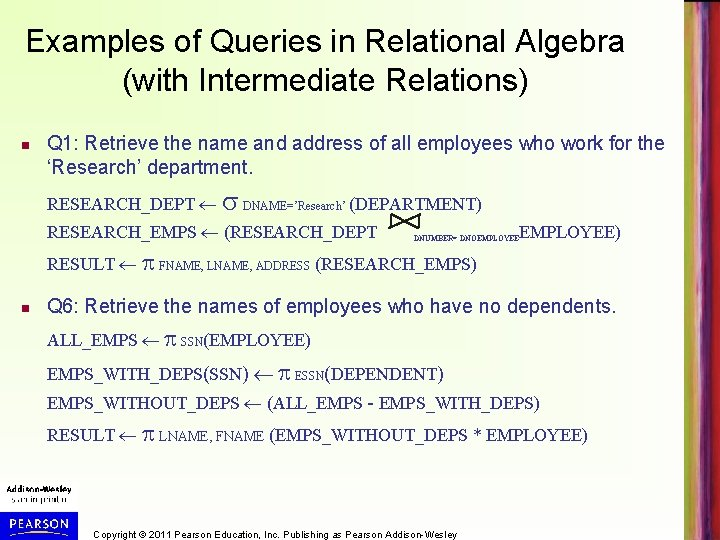Examples of Queries in Relational Algebra (with Intermediate Relations) n Q 1: Retrieve the name and address of all employees who work for the ‘Research’ department. RESEARCH_DEPT DNAME=’Research’ (DEPARTMENT) RESEARCH_EMPS (RESEARCH_DEPT DNUMBER= DNOEMPLOYEE) RESULT FNAME, LNAME, ADDRESS (RESEARCH_EMPS) n Q 6: Retrieve the names of employees who have no dependents. ALL_EMPS SSN(EMPLOYEE) EMPS_WITH_DEPS(SSN) ESSN(DEPENDENT) EMPS_WITHOUT_DEPS (ALL_EMPS - EMPS_WITH_DEPS) RESULT LNAME, FNAME (EMPS_WITHOUT_DEPS * EMPLOYEE) Copyright © 2011 Pearson Education, Inc. Publishing as Pearson Addison-WesleyExamples of Queries in Relational Algebra (Single expressions) As a single expression, these queries become: n Q 1: Retrieve the name and address of all employees who work for the ‘Research’ department. Fname, Lname, Address (σ Dname= ‘Research’ (DEPARTMENT Dnumber=Dno(EMPLOYEE)) n Q 6: Retrieve the names of employees who have no dependents. Lname, Fname(( Ssn (EMPLOYEE) − ρ Ssn ( Essn (DEPENDENT))) ∗ EMPLOYEE) Copyright © 2011 Pearson Education, Inc. Publishing as Pearson Addison-Wesley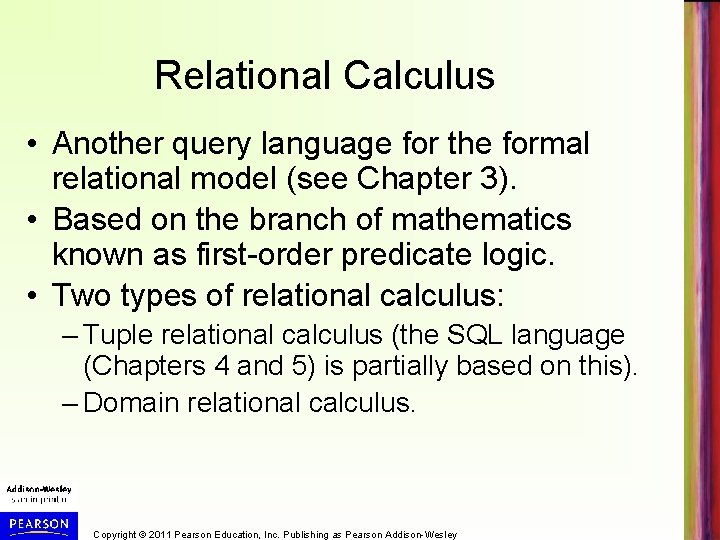Relational Calculus • Another query language for the formal relational model (see Chapter 3). • Based on the branch of mathematics known as first-order predicate logic. • Two types of relational calculus: – Tuple relational calculus (the SQL language (Chapters 4 and 5) is partially based on this). – Domain relational calculus. Copyright © 2011 Pearson Education, Inc. Publishing as Pearson Addison-Wesley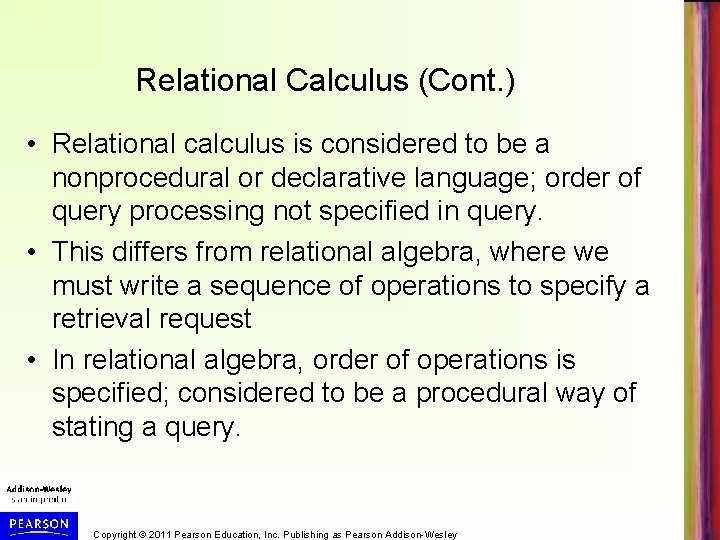Relational Calculus (Cont. ) • Relational calculus is considered to be a nonprocedural or declarative language; order of query processing not specified in query. • This differs from relational algebra, where we must write a sequence of operations to specify a retrieval request • In relational algebra, order of operations is specified; considered to be a procedural way of stating a query. Copyright © 2011 Pearson Education, Inc. Publishing as Pearson Addison-Wesley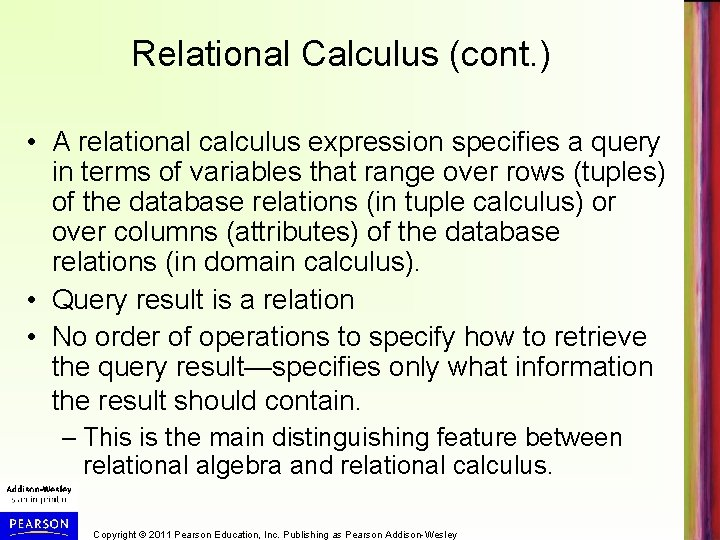Relational Calculus (cont. ) • A relational calculus expression specifies a query in terms of variables that range over rows (tuples) of the database relations (in tuple calculus) or over columns (attributes) of the database relations (in domain calculus). • Query result is a relation • No order of operations to specify how to retrieve the query result—specifies only what information the result should contain. – This is the main distinguishing feature between relational algebra and relational calculus. Copyright © 2011 Pearson Education, Inc. Publishing as Pearson Addison-Wesley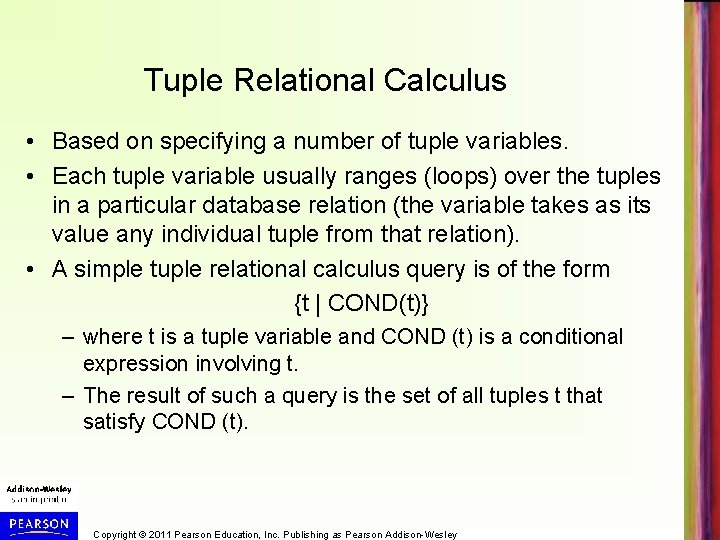Tuple Relational Calculus • Based on specifying a number of tuple variables. • Each tuple variable usually ranges (loops) over the tuples in a particular database relation (the variable takes as its value any individual tuple from that relation). • A simple tuple relational calculus query is of the form {t | COND(t)} – where t is a tuple variable and COND (t) is a conditional expression involving t. – The result of such a query is the set of all tuples t that satisfy COND (t). Copyright © 2011 Pearson Education, Inc. Publishing as Pearson Addison-Wesley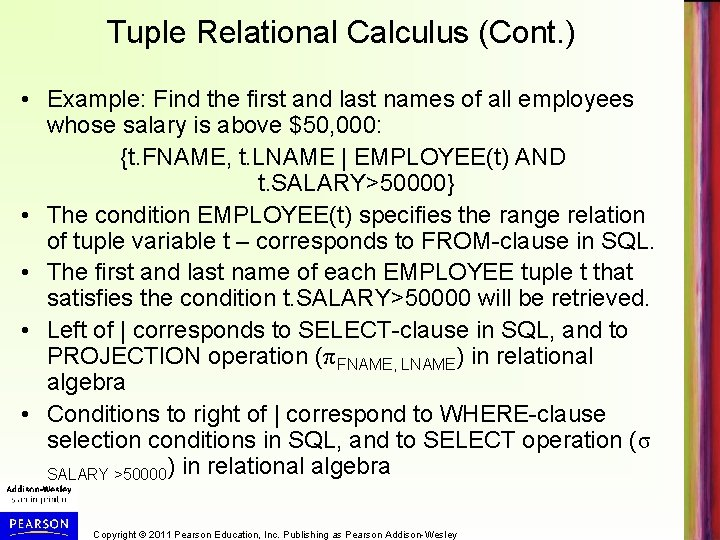Tuple Relational Calculus (Cont. ) • Example: Find the first and last names of all employees whose salary is above \$50, 000: {t. FNAME, t. LNAME | EMPLOYEE(t) AND t. SALARY>50000} • The condition EMPLOYEE(t) specifies the range relation of tuple variable t – corresponds to FROM-clause in SQL. • The first and last name of each EMPLOYEE tuple t that satisfies the condition t. SALARY>50000 will be retrieved. • Left of | corresponds to SELECT-clause in SQL, and to PROJECTION operation (π FNAME, LNAME) in relational algebra • Conditions to right of | correspond to WHERE-clause selection conditions in SQL, and to SELECT operation (σ SALARY >50000) in relational algebra Copyright © 2011 Pearson Education, Inc. Publishing as Pearson Addison-Wesley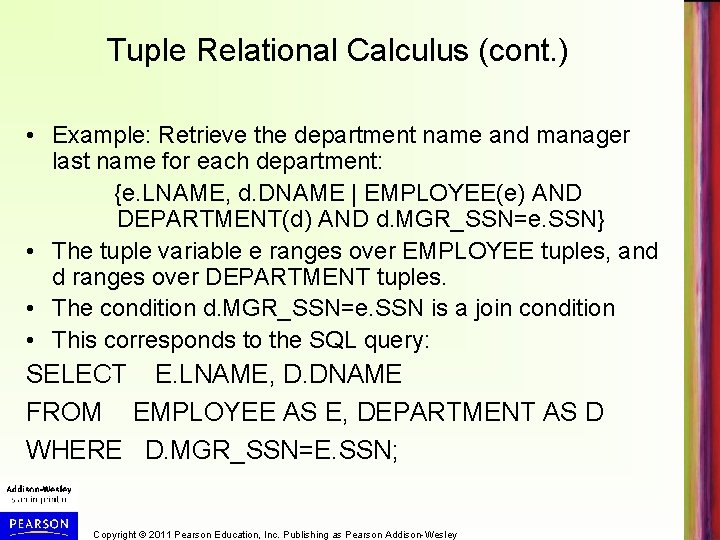Tuple Relational Calculus (cont. ) • Example: Retrieve the department name and manager last name for each department: {e. LNAME, d. DNAME | EMPLOYEE(e) AND DEPARTMENT(d) AND d. MGR_SSN=e. SSN} • The tuple variable e ranges over EMPLOYEE tuples, and d ranges over DEPARTMENT tuples. • The condition d. MGR_SSN=e. SSN is a join condition • This corresponds to the SQL query: SELECT E. LNAME, D. DNAME FROM EMPLOYEE AS E, DEPARTMENT AS D WHERE D. MGR_SSN=E. SSN; Copyright © 2011 Pearson Education, Inc. Publishing as Pearson Addison-WesleyQuery Graph Notation • A data structure to represent a basic tuple relational calculus query expression • Nodes in graph represent tables/aliases and constant values – edges represent selection and join conditions • Example: Retrieve the department name and manager last name for each department: {p. PNUMBER, d. DNUMBER, e. LNAME, e. ADDRESS, e. BDATE | EMPLOYEE(e) AND PROJECT(p) AND DEPARTMENT(d) AND p. PLOCATION='Stafford' AND p. DNUM=d. DNUMBER AND d. MGR_SSN=e. SSN} • Query graph shown on next slide Copyright © 2011 Pearson Education, Inc. Publishing as Pearson Addison-WesleyCopyright © 2011 Pearson Education, Inc. Publishing as Pearson Addison-Wesley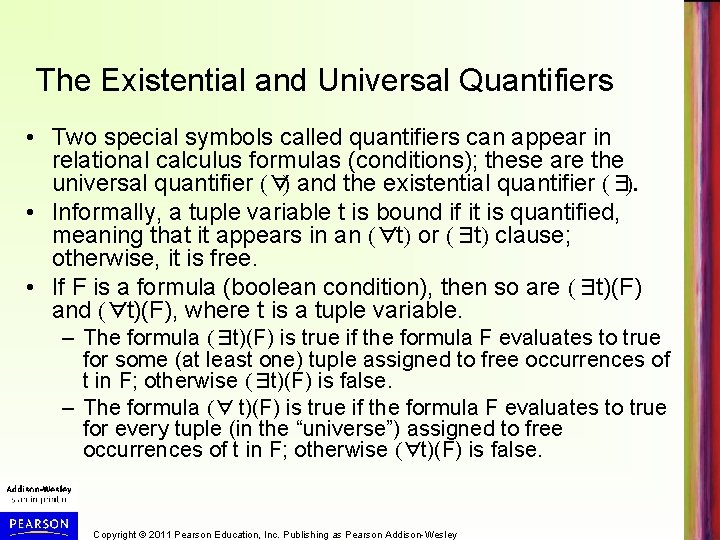The Existential and Universal Quantifiers • Two special symbols called quantifiers can appear in relational calculus formulas (conditions); these are the universal quantifier (∀) and the existential quantifier (∃). • Informally, a tuple variable t is bound if it is quantified, meaning that it appears in an (∀t) or (∃t) clause; otherwise, it is free. • If F is a formula (boolean condition), then so are (∃t)(F) and (∀t)(F), where t is a tuple variable. – The formula (∃t)(F) is true if the formula F evaluates to true for some (at least one) tuple assigned to free occurrences of t in F; otherwise (∃t)(F) is false. – The formula (∀ t)(F) is true if the formula F evaluates to true for every tuple (in the “universe”) assigned to free occurrences of t in F; otherwise (∀t)(F) is false. Copyright © 2011 Pearson Education, Inc. Publishing as Pearson Addison-WesleyExistential and Universal Quantifiers (cont. ) • ∀ is called the universal or “for all” quantifier because every tuple in “the universe of” tuples must make F true to make the quantified formula true. • ∃ is called the existential or “there exists” quantifier because if any (at least one) tuple exists in “the universe of” tuples that makes F, then the quantified formula is true. Copyright © 2011 Pearson Education, Inc. Publishing as Pearson Addison-Wesley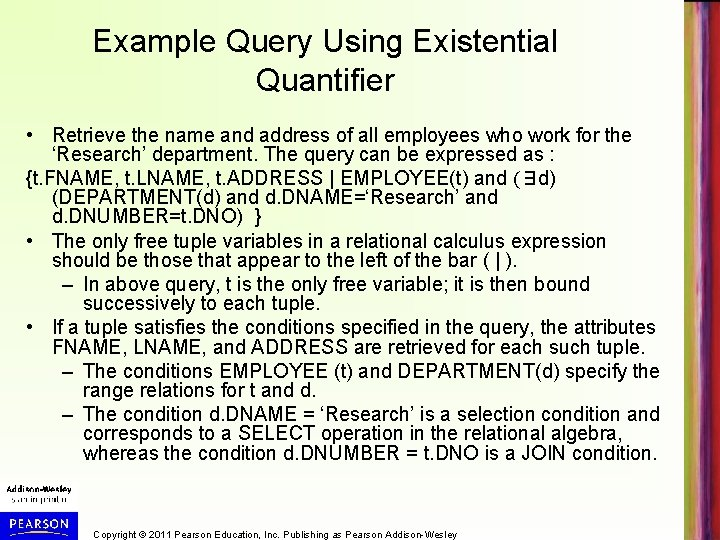Example Query Using Existential Quantifier • Retrieve the name and address of all employees who work for the ‘Research’ department. The query can be expressed as : {t. FNAME, t. LNAME, t. ADDRESS | EMPLOYEE(t) and (∃ d) (DEPARTMENT(d) and d. DNAME=‘Research’ and d. DNUMBER=t. DNO) } • The only free tuple variables in a relational calculus expression should be those that appear to the left of the bar ( | ). – In above query, t is the only free variable; it is then bound successively to each tuple. • If a tuple satisfies the conditions specified in the query, the attributes FNAME, LNAME, and ADDRESS are retrieved for each such tuple. – The conditions EMPLOYEE (t) and DEPARTMENT(d) specify the range relations for t and d. – The condition d. DNAME = ‘Research’ is a selection condition and corresponds to a SELECT operation in the relational algebra, whereas the condition d. DNUMBER = t. DNO is a JOIN condition. Copyright © 2011 Pearson Education, Inc. Publishing as Pearson Addison-WesleyExample Query Using Universal Quantifier • Find the names of employees who work on all the projects controlled by department number 5. The query can be: {e. LNAME, e. FNAME | EMPLOYEE(e) and ( (∀ x)(not(PROJECT(x)) or not(x. DNUM=5) OR ( (∃ w)(WORKS_ON(w) and w. ESSN=e. SSN and x. PNUMBER=w. PNO))))} • Exclude from the universal quantification all tuples that we are not interested in by making the condition true for all such tuples. – The first tuples to exclude (by making them evaluate automatically to true) are those that are not in the relation R of interest. • In query above, using the expression not(PROJECT(x)) inside the universally quantified formula evaluates to true all tuples x that are not in the PROJECT relation. – Then we exclude the tuples we are not interested in from R itself. The expression not(x. DNUM=5) evaluates to true all tuples x that are in the project relation but are not controlled by department 5. • Finally, we specify a condition that must hold on all the remaining tuples in R. ( (∃ w)(WORKS_ON(w) and w. ESSN=e. SSN and x. PNUMBER=w. PNO) Copyright © 2011 Pearson Education, Inc. Publishing as Pearson Addison-Wesley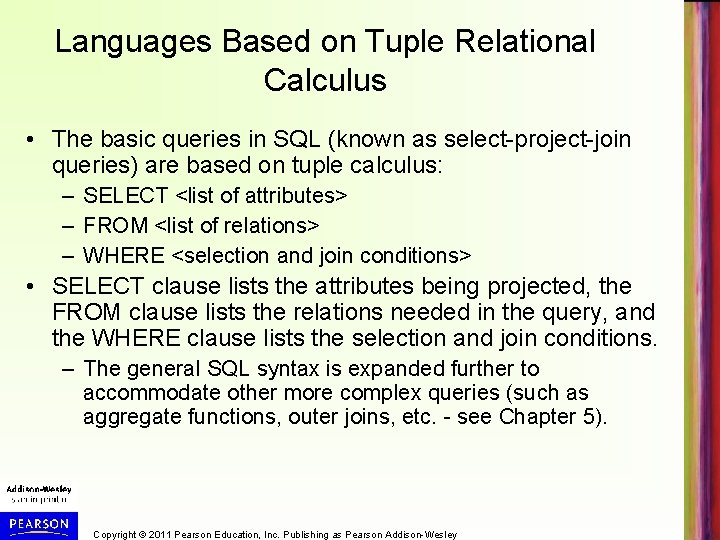Languages Based on Tuple Relational Calculus • The basic queries in SQL (known as select-project-join queries) are based on tuple calculus: – SELECT <list of attributes> – FROM <list of relations> – WHERE <selection and join conditions> • SELECT clause lists the attributes being projected, the FROM clause lists the relations needed in the query, and the WHERE clause lists the selection and join conditions. – The general SQL syntax is expanded further to accommodate other more complex queries (such as aggregate functions, outer joins, etc. - see Chapter 5). Copyright © 2011 Pearson Education, Inc. Publishing as Pearson Addison-WesleyLanguages Based on Tuple Relational Calculus (Cont. ) • Another language based on tuple calculus is the early relational language QUEL, which actually uses range variables as in tuple calculus. Its syntax includes: – RANGE OF <variable name> IS <relation name> • Then it uses – RETRIEVE <list of attributes from range variables> – WHERE <conditions> • This language was proposed in the relational DBMS INGRES (system is currently still supported by Computer Associates – but the QUEL language is no longer there). Copyright © 2011 Pearson Education, Inc. Publishing as Pearson Addison-WesleyThe Domain Relational Calculus • Another variation of relational calculus called the domain relational calculus, or simply, domain calculus is equivalent to tuple calculus and to relational algebra. • The language called QBE (Query-By-Example) that is related to domain calculus was developed almost concurrently to SQL at IBM Research, Yorktown Heights, New York. – Domain calculus was thought of as a way to explain what QBE does. • Domain calculus differs from tuple calculus in the type of variables used in formulas: – Rather than having variables range over tuples, the variables range over single values from domains of attributes. • To form a relation of degree n for a query result, we must have n of these domain variables— one for each attribute. Copyright © 2011 Pearson Education, Inc. Publishing as Pearson Addison-Wesley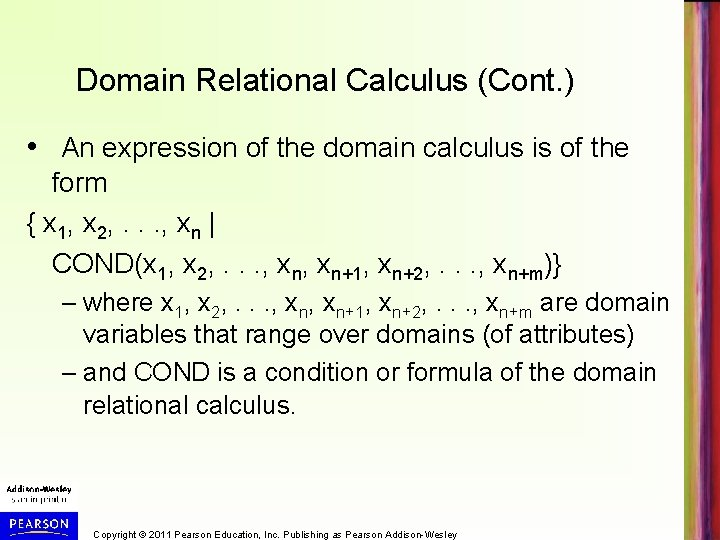Domain Relational Calculus (Cont. ) • An expression of the domain calculus is of the form { x 1, x 2, . . . , xn | COND(x 1, x 2, . . . , xn+1, xn+2, . . . , xn+m)} – where x 1, x 2, . . . , xn+1, xn+2, . . . , xn+m are domain variables that range over domains (of attributes) – and COND is a condition or formula of the domain relational calculus. Copyright © 2011 Pearson Education, Inc. Publishing as Pearson Addison-Wesley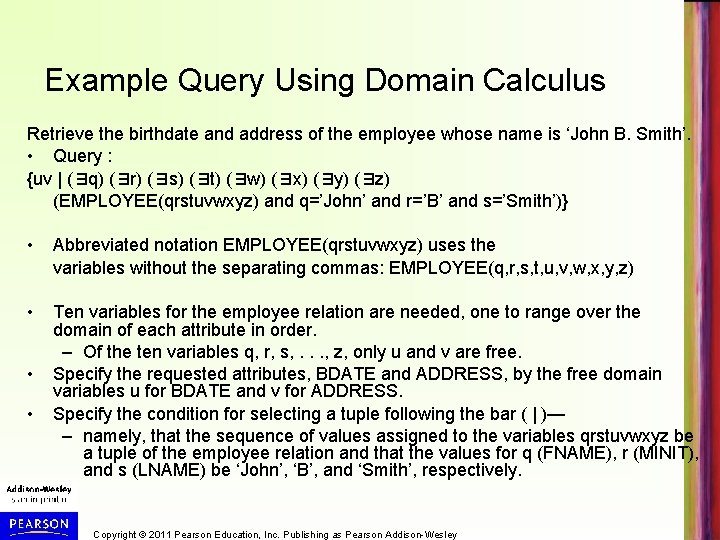Example Query Using Domain Calculus Retrieve the birthdate and address of the employee whose name is ‘John B. Smith’. • Query : {uv | (∃q) (∃r) (∃s) (∃t) (∃w) (∃x) (∃y) (∃z) (EMPLOYEE(qrstuvwxyz) and q=’John’ and r=’B’ and s=’Smith’)} • Abbreviated notation EMPLOYEE(qrstuvwxyz) uses the variables without the separating commas: EMPLOYEE(q, r, s, t, u, v, w, x, y, z) • Ten variables for the employee relation are needed, one to range over the domain of each attribute in order. – Of the ten variables q, r, s, . . . , z, only u and v are free. Specify the requested attributes, BDATE and ADDRESS, by the free domain variables u for BDATE and v for ADDRESS. Specify the condition for selecting a tuple following the bar ( | )— – namely, that the sequence of values assigned to the variables qrstuvwxyz be a tuple of the employee relation and that the values for q (FNAME), r (MINIT), and s (LNAME) be ‘John’, ‘B’, and ‘Smith’, respectively. • • Copyright © 2011 Pearson Education, Inc. Publishing as Pearson Addison-WesleyChapter 6 Summary • Relational Algebra – Unary Relational Operations – Relational Algebra Operations From Set Theory – Binary Relational Operations – Additional Relational Operations – Examples of Queries in Relational Algebra • Relational Calculus – Tuple Relational Calculus – Domain Relational Calculus Copyright © 2011 Pearson Education, Inc. Publishing as Pearson Addison-Wesley